## Introduction to Trigonometry

Trigonometry (from Greek trigonon "triangle" + metron "measure")

Want to learn Trigonometry? Here is a quick summary. Follow the links for more, or go to Trigonometry Index

Trigonometry helps us find angles and distances, and is used a lot in science, engineering, video games, and more!## Right-Angled Triangle

The triangle of most interest is the right-angled triangle . The right angle is shown by the little box in the corner:

Another angle is often labeled θ , and the three sides are then called:

• Opposite : opposite the angle θ
• and the longest side is the Hypotenuse

## Why a Right-Angled Triangle?

Why is this triangle so important?

Imagine we can measure along and up but want to know the direct distance and angle:

Trigonometry can find that missing angle and distance.

Or maybe we have a distance and angle and need to "plot the dot" along and up:

Questions like these are common in engineering, computer animation and more.

## Sine, Cosine and Tangent

The main functions in trigonometry are Sine, Cosine and Tangent

They are simply one side of a right-angled triangle divided by another.

For any angle " θ ":

(Sine, Cosine and Tangent are often abbreviated to sin, cos and tan .)

## Example: What is the sine of 35°?

Using this triangle (lengths are only to one decimal place):

sin(35°) = Opposite Hypotenuse = 2.8 4.9 = 0.57...

The triangle could be larger, smaller or turned around, but that angle will always have that ratio .

Calculators have sin, cos and tan to help us, so let's see how to use them:

## Example: How Tall is The Tree?

We can't reach the top of the tree, so we walk away and measure an angle (using a protractor) and distance (using a laser):

• We know the Hypotenuse
• And we want to know the Opposite

Sine is the ratio of Opposite / Hypotenuse :

sin(45°) = Opposite HypotenuseGet a calculator, type in "45", then the "sin" key:

sin(45°) = 0.7071...

What does the 0.7071... mean? It is the ratio of the side lengths, so the Opposite is about 0.7071 times as long as the Hypotenuse.

We can now put 0.7071... in place of sin(45°):

0.7071... = Opposite Hypotenuse

And we also know the hypotenuse is 20 :

0.7071... = Opposite 20

To solve, first multiply both sides by 20:

20 × 0.7071... = Opposite

Opposite = 14.14m (to 2 decimals)

The tree is 14.14m tall

## Try Sin Cos and Tan

Play with this for a while (move the mouse around) and get familiar with values of sine, cosine and tangent for different angles, such as 0°, 30°, 45°, 60° and 90°.

Also try 120°, 135°, 180°, 240°, 270° etc, and notice that positions can be positive or negative by the rules of Cartesian coordinates , so the sine, cosine and tangent change between positive and negative also.

So trigonometry is also about circles !

## Unit Circle

What you just played with is the Unit Circle .

It is a circle with a radius of 1 with its center at 0.

Because the radius is 1, we can directly measure sine, cosine and tangent.

Here we see the sine function being made by the unit circle:

Note: you can see the nice graphs made by sine, cosine and tangent .

Angles can be in Degrees or Radians . Here are some examples:

## Repeating Pattern

Because the angle is rotating around and around the circle the Sine, Cosine and Tangent functions repeat once every full rotation (see Amplitude, Period, Phase Shift and Frequency ).

When we want to calculate the function for an angle larger than a full rotation of 360° (2 π radians) we subtract as many full rotations as needed to bring it back below 360° (2 π radians):

## Example: what is the cosine of 370°?

370° is greater than 360° so let us subtract 360°

370° − 360° = 10°

cos(370°) = cos(10°) = 0.985 (to 3 decimal places)

And when the angle is less than zero, just add full rotations.

## Example: what is the sine of −3 radians?

−3 is less than 0 so let us add 2 π radians

−3 + 2 π = −3 + 6.283... = 3.283... rad ians

sin(−3) = sin(3.283...) = −0.141 (to 3 decimal places)

## Solving Triangles

Trigonometry is also useful for general triangles, not just right-angled ones .

It helps us in Solving Triangles . "Solving" means finding missing sides and angles.

## Example: Find the Missing Angle "C"

Angle C can be found using angles of a triangle add to 180° :

So C = 180° − 76° − 34° = 70°

We can also find missing side lengths. The general rule is:

When we know any 3 of the sides or angles we can find the other 3 (except for the three angles case)

See Solving Triangles for more details.

## Other Functions (Cotangent, Secant, Cosecant)

Similar to Sine, Cosine and Tangent, there are three other trigonometric functions which are made by dividing one side by another:

## Trigonometric and Triangle Identities

And as you get better at Trigonometry you can learn these:

Enjoy becoming a triangle (and circle) expert!

✅ Unlimited Solutions

➕ Free Bonuses ($1085 value!) Chapter Contents ⊗ ## Trigonometric Functions • 2. Sine, Cosine, Tangent & Reciprocals • 3. Values of Trigonometric Functions • 4. The Right Triangle and Applications • 4a. Trigonometry Revision Summary • 5. Signs of the Trigonometric Functions • 6. Trigonometric Functions of Any Angle • 8. Applications of Radian Measure • 8a. Pulleys • 9. Radians and the Trigonometric Ratios Math Tutoring Need help? Chat with a tutor anytime, 24/7 . Chat Now » Online Trigonometry Solver Solve your trigonometry problem step by step! Online Trigonometry Solver » IntMath Forum Get help with your math queries: IntMath f orum » by M. Bourne ## Why learn about trigonometric functions...?The trig functions are very important in technical subjects like science, engineering, architecture, and even medicine. You will come across them all the time so it's worth learning them well! Surveying is one of the many applications. Road makers, bridge builders and those whose job it is to get buildings in the right place all use trigonometry in their daily work. For more applications and examples of trigonometry in Interactive Mathematics, check out the many Uses of Trigonometry . See also: Math Tips - Trigonometry . In this chapter we start by explaining the basic trigonometric functions using degrees (°), and in the later part of the chapter we will learn about radians and how they are used in trigonometry. ## Related Sections in "Interactive Mathematics" Graphs of Trigonometric Functions , which are really helpful for understanding what is going on in trigonometry. Analytic Trigonometry , which includes double angle formulas, trig ratios of the sum of 2 angles, trigonometric equations and inverse trig equations. Polar Coordinates , which work in much the same way as the topics in this chapter. Derivatives of the Transcendental Functions , which shows how to differentiate sin, cos, tan, csc, sec and cot functions. Integration using Trigonometric Forms , where we see how our knowledge of trigonometry can make calculus easier. Line Spectrum in Fourier Series , which is an advanced application of trigonometry. And of course, don't miss the Uses of Trigonometry summary. ## In this Chapter 1. Angles - the building blocks of trigonometry. In degrees . 2. Sine, Cosine, Tangent & Reciprocals - these fractions are the key for all future trigonometry study. 3. Values of Trigonometric Functions - Why does sin 30° = 0.5 ? 4. The Right Triangle and Applications - includes angle of depressio n and angle of elevation . 5. Signs of the Trigonometric Functions - this is no big deal if you remember how we define the basic ratios. Includes an interactive document for seeing how the ratios of angles larger than 90 degrees work. 6. Trigonometric Functions of Any Angle - don't give up in this section! 7. Radians - the alternative to degrees - and much more useful. 8. Applications of Radian Measure - includes arc length, area of a sector , angular velocity , a game and pulleys . 9. Radians and the Trigonometric Ratios - how trigonometry works when the angle measure is radians . This chapter starts with some reminders about Angles . ## Problem SolverThis tool combines the power of mathematical computation engine that excels at solving mathematical formulas with the power of GPT large language models to parse and generate natural language. This creates math problem solver thats more accurate than ChatGPT, more flexible than a calculator, and faster answers than a human tutor. Learn More. ## Tips, tricks, lessons, and tutoring to help reduce test anxiety and move to the top of the class. Email Address Sign Up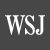The Wall Street Journal ## Finding a Rare Use for Trigonometry After High School Posted: November 9, 2023 | Last updated: November 9, 2023 Mathematician Eugenia Cheng explores the uses of math beyond the classroom. Read more columns here. I recently needed to measure the angle of my computer keyboard, because I currently have it propped up in a rather makeshift manner, and I wanted to find a more permanent solution. I didn’t have a protractor handy, unlike when I was in high school and carried one at all times. But then I realized it was a rare chance to apply some trigonometry. Trigonometry can seem like an endless slew of meaningless formulas that high-schoolers are made to memorize for no obvious reason. Many people opine that they’ve never used it in their daily lives. Nor had I, until now. Here’s how I employed it for my keyboard: I knew that instead of measuring its angle directly with a protractor, I could measure the horizontal distance it took up on my desk and then the vertical distance to its highest point. I don’t remember many trigonometric formulas, but I do know that the gradient, or steepness, of a slope is calculated by dividing the vertical distance by the horizontal distance. From trigonometry you may dimly remember the tan, or tangent, function, which translates between an angle and its associated gradient; if you take the tan of an angle, you get the gradient. Conversely, if you know the gradient you can use the inverse tan function to find the angle. (I can do that on a scientific calculator, but of course most people don’t have one of those handy either; you can also just ask a phone app or the internet for help.) This is also how gradient signs work for roads. It would be hard to use a protractor to measure the angle of slope for a road, so instead the slope is typically indicated by a percentage, which measures how much the vertical distance increases as a proportion of the horizontal distance covered. So a 10% slope means that for every 10 feet you travel horizontally, you go up 10% of that, or 1 foot. It is perhaps counterintuitive, because a 100% slope means that for every foot you travel horizontally, you also travel a foot vertically, which means the angle of the slope would be 45 degrees. But a 50% gradient doesn’t translate to half of that angle: It means that you travel half a foot vertically for every foot horizontally, which translates to an angle of around 27 degrees. Trigonometry used to be much more important than it is now, for example for navigation. Before the days of GPS, sextants were used by sailors to measure the angle of the sun or stars relative to the horizon, and trigonometry could then be used to calculate the ship’s position. Nowadays most of us navigate using a map on our phone rather than a sextant. Trig persists as an important area of study in math class, though the occasions for it—like when you need to find the angles in a triangle based on its sides—are rather limited in daily life. At a deeper level, the value of trig is not so much about solving those specific problems as about the principle of translating concepts from one point of view to another. Angles are proportions of a circle, while lengths and distances are about straight lines. Trig translates between a straight-line point of view and a circular point of view. That’s where its importance lies. In my daily life, trigonometry surprised me by helping to solve my keyboard problem, but the skill of being able to understand the relationship between different points of view applies much more widely.## More for YouAbe Stoklasa, Nashville Musician and Songwriter, Dies at 38Avoid Buying These 10 Cars That Will Likely Break Down After 100K MilesMathematicians Have Found The Ninth Dedekind Number, After 32 Years of SearchingDonald Trump Celebrates 'Gigantic' Legal Victory in Rally SpeechGripped by drought, this island is running out of drinking waterLinda C. Black Horoscopes: November 19Dennis the Menace by H. Ketcham, M. Hamilton & R. FerdinandI Work at Goodwill: Here Are 9 Insider Secrets You Should KnowWatching Ukraine burn through ammo fighting Russia has the US Army rethinking how much it really needs for when war breaks outRon DeSantis says Republicans nominating Donald Trump in 2024 is 'high risk' with a 'low reward'IBM’s new 401(k) strategy could threaten retirement plans for its workersI’m a Mechanic: The Best$100 You Can Spend on Your Car’s Maintenance‘Extremely unusual’: CNN data reporter on new polling ahead of 2024 electionSuzanne Shepherd, 'Sopranos' and 'Goodfellas' actress, dies at 89Ukraine says it has pushed Russian forces back up to 5 miles from the banks of Dnipro river, in first possible counteroffensive success10 Countries To Live Outside the U.S. That Are So Cheap You Could Quit Your JobMy ex-husband paid the mortgage on our home for 20 years. Do I get half if he sells?Israel and Hamas war reveals ugly antisemitism in America. It's scary to see.Jan. 6 Footage of Pelosi Speaking With Pence Sparks Conspiracy TheoryHi & Lois by Chance Browne and Eric Reaves

## Trigonometric functions

Trigonometric functions are functions related to an angle . There are six trigonometric functions: sine, cosine, tangent and their reciprocals cosecant, secant, and cotangent, respectively. Sine, cosine, and tangent are the most widely used trigonometric functions. Their reciprocals, though used, are less common in modern mathematics. Trigonometric functions are also called circular functions.

## 6 trig functions

The table below shows the six trigonometric function values for the specified angles in both degrees and radians.

## Trigonometric function definitions

There are two main ways in which trigonometric functions are typically discussed: in terms of right triangles and in terms of the unit circle . The right-angled triangle definition of trigonometric functions is most often how they are introduced, followed by their definitions in terms of the unit circle.

## Right triangle definition

The output of a trigonometric function is a ratio of the lengths of two sides of a right triangle. Consider an angle θ as one angle in a right triangle. The following are the definitions of the trigonometric functions. These functions are often written in their abbreviated forms.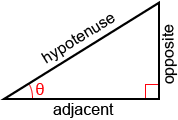The terms used to describe the sides of a right triangle are the hypotenuse, the adjacent side, and the opposite side, as shown in the figure above.

• Adjacent: the side next to θ that is not the hypotenuse
• Opposite: the side opposite θ.
• Hypotenuse: the longest side of the triangle opposite the right angle.

A common method for remembering the above relationships is through use of the mnemonic “soh cah toa.” The s, c, and t stand for sine, cosine, and tangent, and o, a, and h stand for opposite, adjacent, and hypotenuse.

Cosecant, secant, and cotangent are the reciprocals of sine, cosine, and tangent, respectively. As such, as long as we remember the definitions of sine, cosine, and tangent, we can take their reciprocals to determine the definitions of cosecant, secant, and cotangent.

Find the values of the six trigonometric given the right triangle below.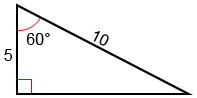The hypotenuse of the triangle is 10, and the adjacent side has a length of 5. Using the Pythagorean theorem , we can find the length of the third, opposite, side:

5 2 + b 2 = 10 2

25 + b 2 = 100

Now that we know all the sides of the triangle with reference to the angle, 60°, we can plug them into the trigonometric functions as defined above:

## Unit circle definition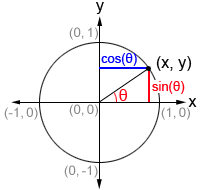Given a point (x, y) on the unit circle, we can form a right triangle, as shown in the figure. In such a triangle, the hypotenuse is the radius of the unit circle, or 1. θ is the angle formed between the initial side of an angle along the x-axis and the terminal side of the angle formed by rotating the ray either clockwise or counterclockwise. The terminal side of the angle is the hypotenuse of the right triangle and is the radius of the unit circle. Therefore, it always has a length of 1. The point at which the terminal side of the angle intersects the unit circle has an x-value of cos⁡(θ) and y-value of sin⁡(θ).

Thus, on the unit circle, cosine and sine can be defined as:

For tan⁡(θ), x cannot be equal to 0.

Cosecant, secant, and cotangent are the reciprocals of sine, cosine, and tangent respectively, and are defined as:

The values of the trigonometric functions can also be represented by the lengths of the line segments in a coordinate plane with a unit circle as show in the diagram below.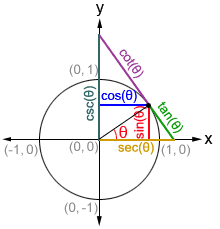## Trigonometric function values for the special angles

The values of trigonometric functions can be found through the coordinate values of the intersections on a unit circle. While we can find the value of any of the trigonometric functions for any value of θ, there are some angles that are more frequently used in trigonometry and worth memorizing.

## Reference angles

Acute angles in the first quadrant can be used to determine the values of trigonometric functions of angles in other quadrants. Below is a table of the values of the sine, cosine, and tangent functions for special angles in the first quadrant , referred to as reference angles.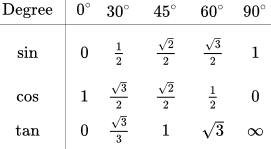These angles are called reference angles since we will reference their values to determine other values. It is always the smallest angle (with reference to the x-axis) that can be made from the terminal side of an angle. The figures below shows an angle θ and its reference angle θ' in a quadrant other than the first quadrant.

Below is a table showing the signs of the 6 trigonometric functions in each quadrant.

Once we determine the reference angle, we can determine the value of the trigonometric functions in any of the other quadrants by applying the appropriate sign to their value for the reference angle.

Use reference angles to find the values of cos(150°) and sin(315°).

## Trigonometric functions are periodic functions

A periodic function is a function, f, in which some positive value, p, exists such that

f(x+p) = f(x)

for all x in the domain of f, p is the smallest positive number for which f is periodic, and is referred to as the period of f.

All 6 trigonometric functions are periodic functions. No matter what point we start at on the unit circle, if we travel a distance of 2π (360°) along the unit circle from that point, we will arrive back at our starting point, which indicates that the trigonometric function have the same value at the angle. This means the trigonometric functions repeat their values.

The sine, cosine, cosecant, and secant functions have a period of 2π. The tangent and cotangent functions have a period of π.

sin(θ+2π) = sin (θ)

cos(θ+2π) = cos (θ)

csc(θ+2π) = csc (θ)

sec(θ+2π) = sec (θ)

tan(θ+π) = tan (θ)

cot(θ+π) = cot (θ)

## Trigonometric functions are odd or even

An odd function is a function in which -f(x)=f(-x). It has symmetry about the origin. An even function is a function in which f(x)=f(-x) meaning that reflecting the graph across the y-axis will yield the same graph. Of the 6 trigonometric functions, sine, tangent, cosecant, and cotangent are odd functions. Cosine and secant are even functions. Therefore:

## Inverse trigonometric functions

The inverse trigonometric functions are the inverse functions of the trigonometric functions. Specifically, they are arcsine, arccosine, arctangent, arccosecant, arcsecant, and arctangent. The input of the inverse trigonometric functions is an angle's trigonometric ratios, and its output is the angle:

The inverse trigonometric functions are also written as sin -1 ⁡(x), cos -1 ⁡(x), tan -1 ⁡(x), csc -1 ⁡(x), sec -1 ⁡(x), and cot -1 ⁡(x).

Given sin(30°) = 0.5, what is arcsin(0.5)?

arcsin(0.5) = 30°

Note: this only provides the result in the first quadrant. If consider other quadrants, arcsin(0.5) is also equal to 150°. Also, since arcsin is a periodic function, to account for all the possible values of arcsine, we need to account for its periodicity. The solution is therefore

30°+n×360° or 150°+n×360°

where n is an integer. When using the other inverse trigonometric functions, their periodicity also needs to be taken into account to determine all the solutions.

## Graphs of the trigonometric functions

The figure below shows the graphs of several periods of the six trigonometric functions. Refer to the sine , cosine , and tangent pages for an in-depth explanation on how to graph trigonometric functions that have undergone certain transformations (the same explanations apply to cosecant, secant, and cotangent, with minor differences).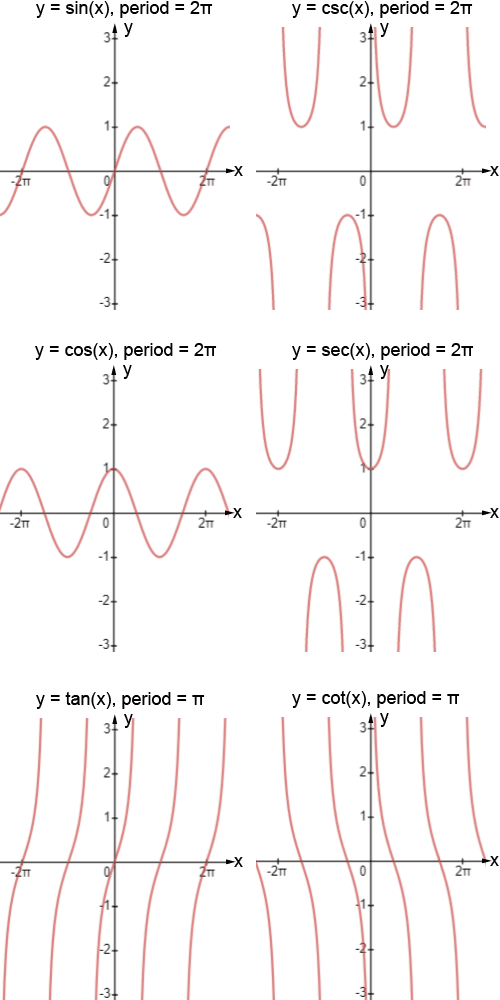## Trigonometric Functions

Trigonometric functions are the basic six functions that have a domain input value as an angle of a right triangle, and a numeric answer as the range. The trigonometric function (also called the 'trig function') of f(x) = sinθ has a domain, which is the angle θ given in degrees or radians, and a range of [-1, 1]. Similarly we have the domain and range from all other functions. Trigonometric functions are extensively used in calculus, geometry, algebra.

Here in the below content, we shall aim at understanding the trigonometric functions across the four quadrants, their graphs, the domain and range, the formulas, and the differentiation, integration of trigonometric functions. We will solve a few examples using these six trig functions for a better understanding of them and their applications.

## What are Trigonometric Functions?

There are six basic trigonometric functions used in Trigonometry. These functions are trigonometric ratios. The six basic trigonometric functions are sine function , cosine function , secant function, co-secant function, tangent function , and co-tangent function. The trigonometric functions and identities are the ratio of sides of a right-angled triangle. The sides of a right triangle are the perpendicular side, hypotenuse, and base, which are used to calculate the sine, cosine, tangent, secant , cosecant , and cotangent values using trigonometric formulas.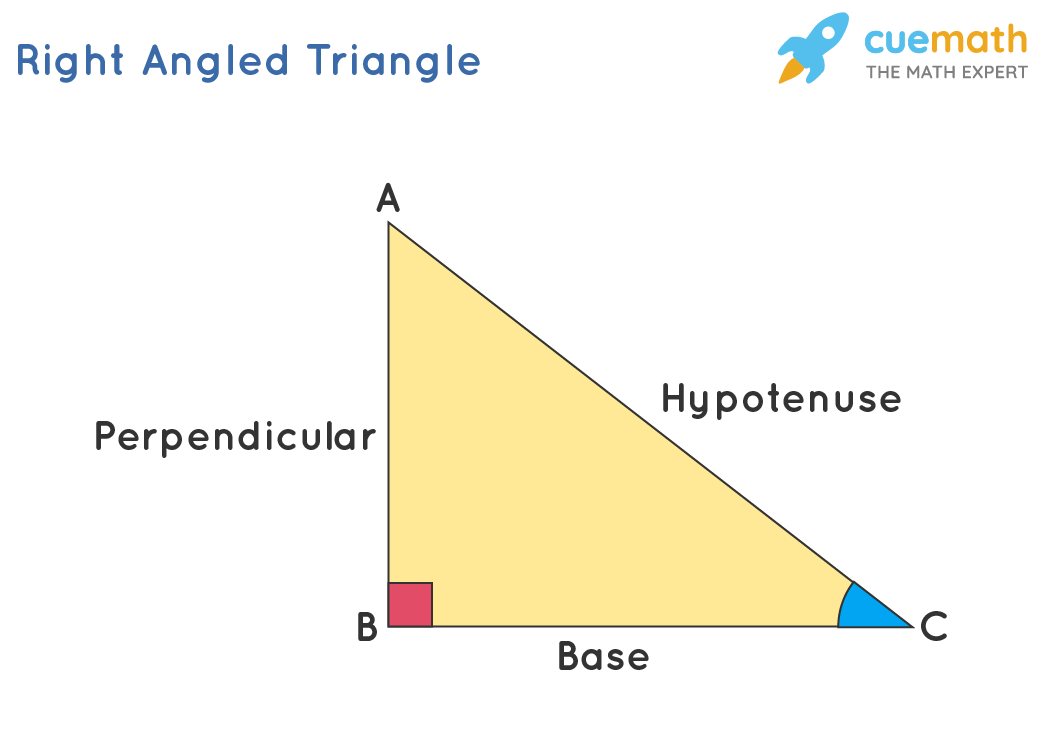## Trigonometric Functions Formulas

We have certain formulas to find the values of the trig functions using the sides of a right-angled triangle. To write these formulas, we use the abbreviated form of these functions. Sine is written as sin, cosine is written as cos, tangent is denoted by tan, secant is denoted by sec, cosecant is abbreviated as cosec, and cotangent is abbreviated as cot. The basic formulas to find the trigonometric functions are as follows:

• sin θ = Perpendicular/Hypotenuse
• cos θ = Base/Hypotenuse
• tan θ = Perpendicular/Base
• sec θ = Hypotenuse/Base
• cosec θ = Hypotenuse/Perpendicular
• cot θ = Base/Perpendicular

As we can observe from the above-given formulas, sine and cosecant are reciprocals of each other. Similarly, the reciprocal pairs are cosine and secant, and tangent and cotangent.

## Trigonometric Functions Values

The trigonometric functions have a domain θ, which is in degrees or radians . Some of the principal values of θ for the different trigonometric functions are presented below in a table . These principal values are also referred to as standard values of trig functions at specific angles and are frequently used in calculations. The principal values of trigonometric functions have been derived from a unit circle. These values also satisfy all the trigonometric formulas .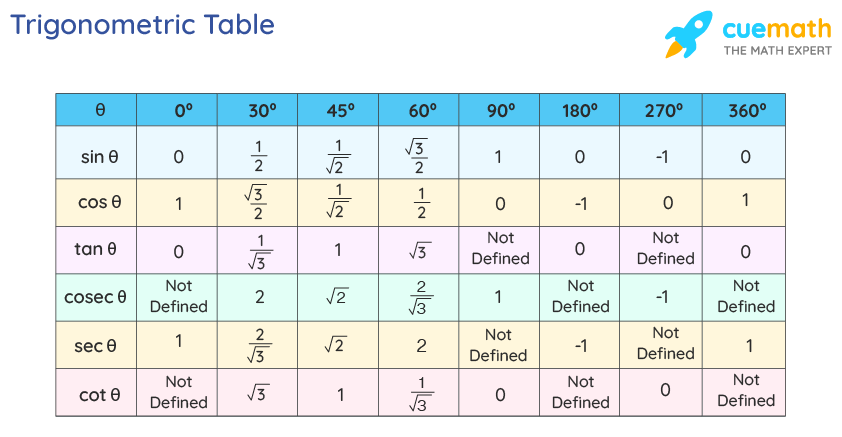## Trig Functions in Four Quadrants

The angle θ is an acute angle (θ < 90°) and is measured with reference to the positive x-axis, in the anticlockwise direction. Further, these trig functions have different numeric signs (+ or -) in the different quadrants, which are based on the positive or negative axis of the quadrant. The trigonometric functions of Sinθ, Cosecθ are positive in quadrants I and II, and are negative in quadrants III and IV. All the trigonometric functions have a positive range in the first quadrant. The trigonometric functions Tanθ, Cotθ are positive only in Quadrants I and III, and the trigonometric ratios of Cosθ, Secθ are positive only in quadrants I and IV.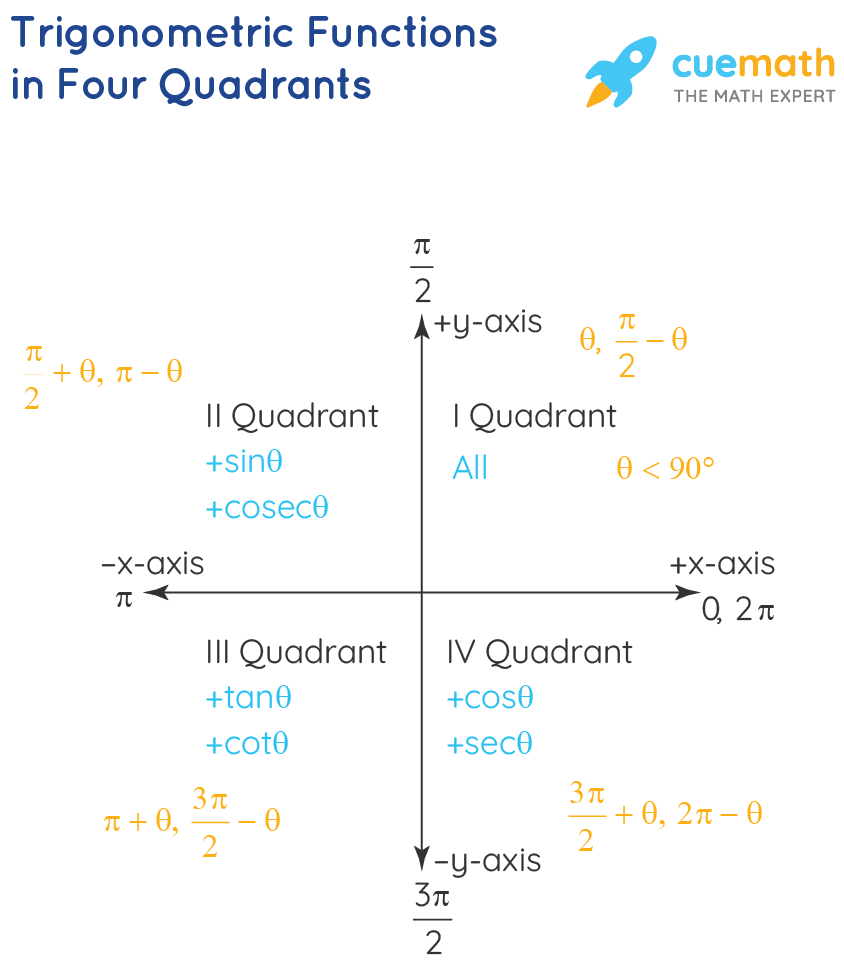The trigonometric functions have values of θ, (90° - θ) in the first quadrant. The cofunction identities provide the interrelationship between the different complementary trigonometric functions for the angle (90° - θ).

• sin(90°−θ) = cos θ
• cos(90°−θ) = sin θ
• tan(90°−θ) = cot θ
• cot(90°−θ) = tan θ
• sec(90°−θ) = cosec θ
• cosec(90°−θ) = sec θ

The domain θ value for different trigonometric function in the second quadrant is (π/2 + θ, π - θ), in the third quadrant is (π + θ, 3π/2 - θ), and in the fourth quadrant is (3π/2 + θ, 2π - θ). For π/2, 3π/2 the trigonometric values changes as their complementary ratios such as Sinθ⇔Cosθ, Tanθ⇔Cotθ, Secθ⇔Cosecθ. For π, 2π the trigonometric values remain the same. The changing trigonometric ratios in different quadrants and angles can be understood from the below table.

## Trigonometric Functions Graph

The graphs of trigonometric functions have the domain value of θ represented on the horizontal x-axis and the range value represented along the vertical y-axis. The graphs of Sinθ and Tanθ pass through the origin and the graphs of other trigonometric functions do not pass through the origin. The range of Sinθ and Cosθ is limited to [-1, 1]. The range of infinite values is presented as drawn beside the dotted lines.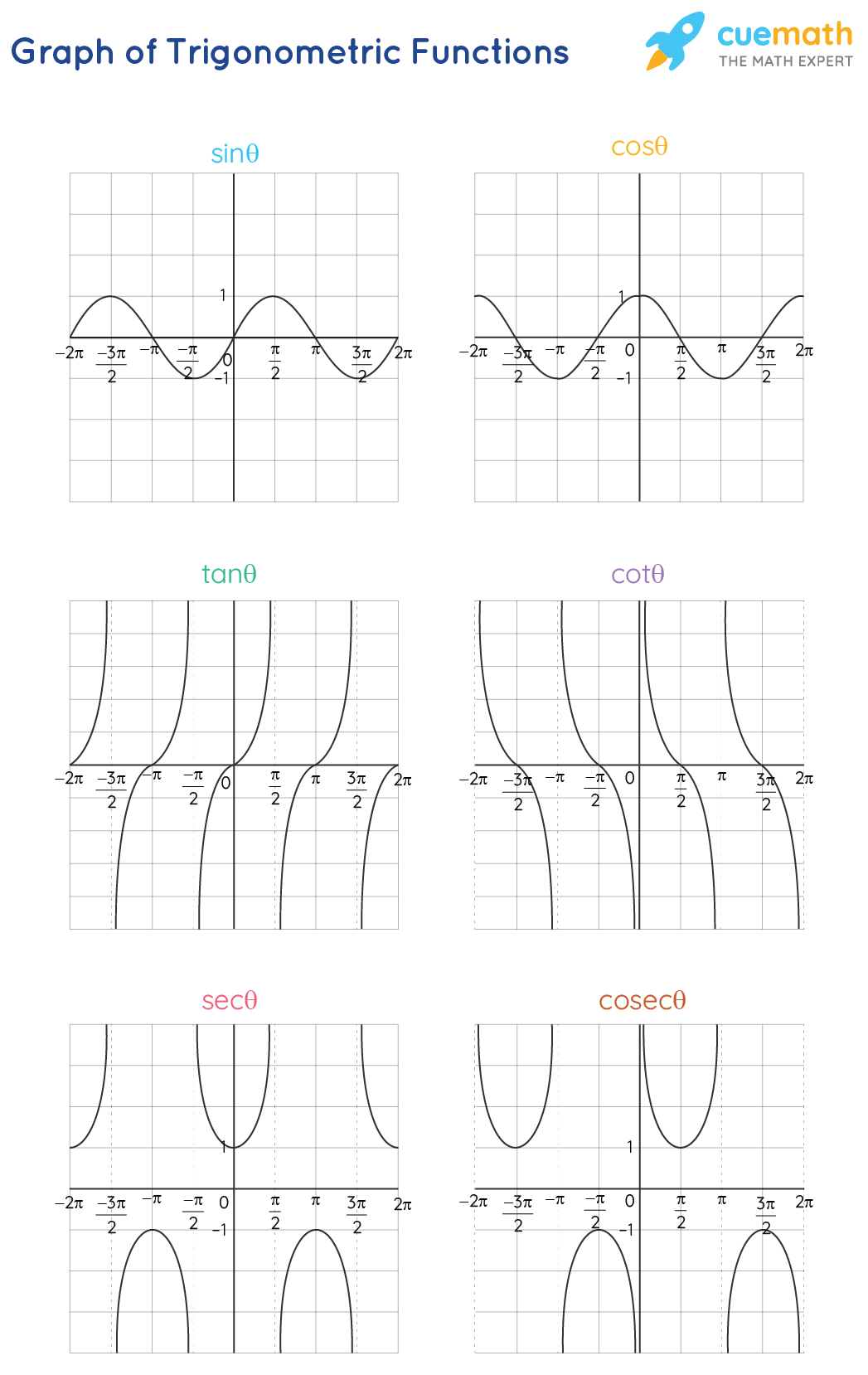## Domain and Range of Trigonometric Functions

The value of θ represents the domain of the trigonometric functions and the resultant value is the range of the trigonometric function. The domain values of θ are in degrees or radians and the range is a real number value. Generally, the domain of the trigonometric function is a real number value, but in certain cases, a few angle values are excluded because it results in a range as an infinite value. The trigonometric function are periodic functions . The below table presents the domain and range of the six trigonometric functions .

## Trigonometric Functions Identities

The trigonometric functions identities are broadly divided into reciprocal identities, Pythagorean formulas, sum and difference of trig functions identities, formulas for multiple and sub-multiple angles, sum and product of identities. All of these below formulas can be easily derived using the ratio of sides of a right-angled triangle. The higher formulas can be derived by using the basic trigonometric function formulas. Reciprocal identities are used frequently to simplify trigonometric problems.

## Reciprocal Identities

• cosec θ = 1/sin θ
• sec θ = 1/cos θ
• cot θ = 1/tan θ
• sin θ = 1/cosec θ
• cos θ = 1/sec θ
• tan θ = 1/cot θ

## Pythagorean Identities

• Sin 2 θ + Cos 2 θ = 1
• 1 + Tan 2 θ = Sec 2 θ
• 1 + Cot 2 θ = Cosec 2 θ

## Sum and Difference Identities

• sin(x+y) = sin(x)cos(y) + cos(x)sin(y)
• cos(x+y) = cos(x)cos(y) – sin(x)sin(y)
• tan(x+y) = (tan x + tan y)/ (1−tan x tan y)
• sin(x–y) = sin(x)cos(y) – cos(x)sin(y)
• cos(x–y) = cos(x)cos(y) + sin(x)sin(y)
• tan(x−y) = (tan x–tan y)/ (1+tan x tan y)

## Half-Angle Identities

• sin A/2 = ±√[(1 - cos A) / 2]
• cos A/2 = ±√[(1 + cos A) / 2]
• tan A/2 = ±√[(1 - cos A) / (1 + cos A)] (or) sin A / (1 + cos A) (or) (1 - cos A) / sin A

## Double Angle Identities

• sin(2x) = 2sin(x) cos(x) = [2tan x/(1+tan 2 x)]
• cos(2x) = cos 2 (x)–sin 2 (x) = [(1-tan 2 x)/(1+tan 2 x)]
• cos(2x) = 2cos 2 (x)−1 = 1–2sin 2 (x)
• tan(2x) = [2tan(x)]/ [1−tan 2 (x)]
• cot(2x) = [cot 2 (x) - 1]/[2cot(x)]
• sec (2x) = sec 2 x/(2-sec 2 x)
• cosec (2x) = (sec x. cosec x)/2

## Triple Angle Identities

• Sin 3x = 3sin x – 4sin 3 x
• Cos 3x = 4cos 3 x - 3cos x
• Tan 3x = [3tanx-tan 3 x]/[1-3tan 2 x]

## Product identities

• 2sinx⋅cosy=sin(x+y)+sin(x−y)
• 2cosx⋅cosy=cos(x+y)+cos(x−y)
• 2sinx⋅siny=cos(x−y)−cos(x+y)

## Sum of Identities

• sinx+siny=2sin((x+y)/2) . cos((x−y)/2)
• sinx−siny=2cos((x+y)/2) . sin((x−y)/2)
• cosx+cosy=2cos((x+y)/2) . cos((x−y)/2)
• cosx−cosy=−2sin((x+y)/2 . sin((x−y)/2)

## Inverse Trigonometric Functions

Inverse trigonometric functions are the inverse ratio of the basic trigonometric ratios . Here the basic trigonometric function of Sin θ = x, can be changed to Sin -1 x = θ. Here x can have values in whole numbers, decimals , fractions , or exponents . For θ = 30° we have θ = Sin -1 (1/2). All the trigonometric formulas can be transformed into inverse trigonometric function formulas.

Arbitrary Values: The inverse trigonometric ratio formula for arbitrary values is applicable for all the six trigonometric functions. For the inverse trigonometric functions of sine, tangent, cosecant, the negative of the values are translated as the negatives of the function. And for functions of cosecant, secant, cotangent, the negatives of the domain are translated as the subtraction of the function from the π value.

• Sin -1 (-x) = -Sin -1 x
• Tan -1 (-x) = -Tan -1 x
• Cosec -1 (-x) = -Cosec -1 x
• Cos -1 (-x) = π - Cos -1 x
• Sec -1 (-x) = π - Sec -1 x
• Cot -1 (-x) = π - Cot -1 x

The inverse trigonometric functions of reciprocal and complementary functions are similar to the basic trigonometric functions. The reciprocal relationship of the basic trigonometric functions, sine-cosecant, cos-secant, tangent-cotangent, can be interpreted for the inverse trigonometric functions. Also the complementary functions, since-cosine, tangent-cotangent, and secant-cosecant can be interpreted into:

Reciprocal Functions: The inverse trigonometric formula of inverse sine, inverse cosine, and inverse tangent can also be expressed in the following forms.

• Sin -1 x = Cosec -1 1/x
• Cos -1 x = Sec -1 1/x
• Tan -1 x = Cot -1 1/x

Complementary Functions: The complementary functions of sine-cosine, tangent-cotangent, secant-cosecant, sum up to π/2.

• Sin -1 x + Cos -1 x = π/2
• Tan -1 x + Cot -1 x = π/2
• Sec -1 x + Cosec -1 x = π/2

## Trigonometric Functions Derivatives

The differentiation of trigonometric functions gives the slope of the tangent of the curve. The differentiation of Sinx is Cosx and here on applying the x value in degrees for Cosx we can obtain the slope of the tangent of the curve of Sinx at a particular point. The formulas of differentiation of trigonometric functions are useful to find the equation of a tangent, normal, to find the errors in calculations.

• d/dx. Sinx = Cosx
• d/dx. Cosx = -Sinx
• d/dx. Tanx = Sec 2 x
• d/dx. Cotx = -Cosec 2 x
• d/dx.Secx = Secx.Tanx
• d/dx. Cosecx = - Cosecx.Cotx

## Integration of Trigonometric Function

The integration of trigonometric functions is helpful to find the area under the graph of the trigonometric function. Generally, the area under the graph of the trigonometric function can be calculated with reference to any of the axis lines and within a defined limit value. The integration of trigonometric functions is helpful to generally find the area of irregularly shaped plane surfaces.

• ∫ cosx dx = sinx + C
• ∫ sinx dx = -cosx + C
• ∫ sec 2 x dx = tanx + C
• ∫ cosec 2 x dx = -cotx + C
• ∫ secx.tanx dx = secx + C
• ∫ cosecx.cotx dx = -cosecx + C
• ∫ tanx dx = log|secx| + C
• ∫ cotx.dx = log|sinx| + C
• ∫ secx dx = log|secx + tanx| + C
• ∫ cosecx.dx = log|cosecx - cotx| + C

Related Topics

• Trigonometry
• Sum to Product Formulas
• Algebraic Identities

## Solved Examples on Trigonometric Functions

Example 1: Find the value of Sin75°.

The aim is to find the value of Sin75°.

Her we can use the formula Sin(A + B) = SinA.CosB + CosA.SinB.

Here we have A = 30° and B = 45°

Sin 75° = Sin(30° + 45°)

= Sin30°.Cos45° + Cos30°.Sin45°

= (1/2) (1/√2) + (√3/2) (1/√2)

= 1/2√2 + √3/2√2

= (√3 + 1) / 2√2

Answer: Sin75° = (√3 + 1) / 2√2

Example 2: Find the value of the trigonometric functions, for the given value of 12Tanθ = 5.

Given 12Tanθ = 5, and we have Tanθ = 5/12

Tanθ = Perpendicular/Base = 5/12

Applying the Pythagorean theorem we have:

Hypotenuse 2 = Perpendicular 2 + Base 2

Hyp 2 = 12 2 + 5 2

Hence the other trigonometric functions are as follows.

Sinθ = Perp/Hyp = 5/13

Cosθ = Base/Hyp = 12/13

Cotθ = Base/Perp = 12/5

Secθ = Hyp/Base = 13/12

Cosecθ = Hyp/Perp = 13/5

Example 3: Find the value of the product of the six trigonometric functions.

Solution: We know that cosec x is the reciprocal of sin x and sec x is the reciprocal of cos x. Also, tan x can be written as the ratio of sin x and cos x, cot x can be written as the ratio of cos x and sin x. So, we have

sinx × cosx × tanx × cotx × secx × cosecx = sinx × cosx × (sinx/cosx) × (cosx/sinx) × (1/cosx) × (1/sinx)

= (sinx × cosx) / (sinx × cosx) × (sinx/cosx) × (cosx/sinx)

= 1 × 1

Answer: Product of the six trigonometric functions is equal to 1.

go to slide go to slide go to slideBook a Free Trial Class

## Practice Questions on Trigonometric Functions

go to slide go to slide

## FAQs on Trigonometric Functions

What are the six trigonometric functions.

The trigonometric functions are the result of the ratio of the sides of the right angles triangle. For the three sides of the triangle as hypotenuse, base, altitude, and for the angle between the hypotenuse and the base being θ, the value of the six trigonometric ratios is as follows.

• Sinθ = Altitude/Hypotenuse
• Cosθ = Base/Hypotenuse
• Tanθ = Altitude/Base
• Cotθ = Base/Altitude
• Secθ = Hypotenuse/Base
• Cosecθ = Hypotenuse/Altitude

## How Do you Find Trigonometric Functions?

The trigonometric functions are the ratio of the sides of a right-angled triangle . Further, we also apply the Pythagorean rule of Hypotenuse 2 = Altitude 2 + Base 2 . Also, the trigonometric functions have different values for different angle values between the hypotenuse and the base of the right triangle.

## What is the Domain and Range of Trigonometric Functions?

The domain of a trigonometric function is the value of θ in Sinθ, and the range is the final numeric value of Sinθ. This concept can be similarly applied to all the other trigonometric functions. Further, the domain values can be any angular values, but here we have the principal values of angles as 0°, 30°, 45°, 60°, 90°. And the range is the highest and the lowest values which are obtained. It is [-1, 1] for sinθ, cosθ, and it is (-∞, +∞) for tanθ, cotθ.

## What is the Result of Multiplying Six Trigonometric Functions?

The result of the multiplication of the six trigonometric functions is as follows. Sinθ.Cosθ.Tanθ.Cotθ.Secθ.Cosecθ = Sinθ.Cosθ.Sinθ/Cosθ.Cosθ/Sinθ.1/Cosθ.1/Sinθ = 1.## What is the General Solution of Trigonometric Function of Sinx?

The general solution of Sinx is nπ + (-1) n x. This represents all the higher angle values of Sinx. For x = π/3 we have the higher values of x as 2π/3, 7π3, and the general solution of x is nπ +(-1) n π/3.

## What is the General Solution of the Trigonometric Function of Cosx?

The general solution of Cosx is 2nπ + x. This general solution represents all the higher angle values of Cosx. For x = π/4, the higher values of x are 7π/4, 9π/4, and the general solution of x is 2nπ + π/4.

## What is the General Solution of the Trig Function of Tanx?

The general solution of Tanx is nπ + x. The general solution represents all the higher angle values of Tanx. For x = π/6, the higher values of x are 7π/6, 13π/6, and the general solution of x is nπ + π/6.

## How to Differentiate Trigonometric Functions?

The differentiation of trigonometric function results in the slope of the tangent to the curve of the trigonometric function. The differentiation of sinx results in cosx, which by substituting the value of x in degrees gives the slope value of the tangent to the curve of sinx. The differentiation is calculated using the first principle of derivatives. Further, we have the differentiation of the six trigonometric functions as follows.

## What are the Applications of Trigonometric Functions?

The trigonometric functions have numerous applications in calculus coordinate geometry algebra. The slope of a line , the normal form of the equation of a lie, parametric coordinates of a parabola , ellipse , hyperbola, are all calculated and represented using trigonometric functions. The trigonometric functions can be used to find the height of a tree, for the given distance of the tree from the point of observation. Further, the trigonometric functions are extensively used in astronomy, to find distances of stars and celestial bodies, with the help of the given angle value.

## Definition of trig

(Entry 1 of 2)

Definition of trig  (Entry 2 of 2)

• uncluttered
• well-groomed

## Examples of trig in a Sentence

These examples are programmatically compiled from various online sources to illustrate current usage of the word 'trig.' Any opinions expressed in the examples do not represent those of Merriam-Webster or its editors. Send us feedback about these examples.

## Word History

by shortening

Middle English, trusty, nimble, of Scandinavian origin; akin to Old Norse tryggr faithful; akin to Old English trēowe faithful — more at true entry 1

circa 1878, in the meaning defined above

1513, in the meaning defined at sense 1

## Dictionary Entries Near trig

Cite this entry.

“Trig.” Merriam-Webster.com Dictionary , Merriam-Webster, https://www.merriam-webster.com/dictionary/trig. Accessed 19 Nov. 2023.

## Kids Definition

Kids definition of trig.

Subscribe to America's largest dictionary and get thousands more definitions and advanced search—ad free!## Can you solve 4 words at once?

Word of the day.

See Definitions and Examples »

Get Word of the Day daily email!

## Games & Quizzes• Math Article

## Trigonometry

Trigonometry is one of the important branches in the history of mathematics  that deals with the study of the relationship between the sides and angles of a   right-angled triangle. This concept is given by the Greek mathematician Hipparchus. In this article, we are going to learn the basics of trigonometry such as trigonometry functions, ratios, trigonometry table, formulas and many solved examples.

## What is Trigonometry?

Trigonometry is one of the most important branches in mathematics that finds huge application in diverse fields. The branch called “Trigonometry” basically deals with the study of the relationship between the sides and angles of the right-angle triangle.  Hence, it helps to find the missing or unknown angles or sides of a right triangle using the trigonometric formulas, functions or trigonometric identities. In trigonometry, the angles can be either measured in degrees or radians. Some of the most commonly used trigonometric angles for calculations are 0°, 30°, 45°, 60° and 90°.

Trigonometry is further classified into two sub-branches. The two different types of trigonometry are:

• Plane Trigonometry
• Spherical Trigonometry

In this article, let us discuss the six important trigonometric functions, ratios, trigonometry table, formulas and identities which helps to find the missing angles or sides of a right triangle.

## Trigonometry Ratios-Sine, Cosine, Tangent

The trigonometric ratios of a triangle are also called the trigonometric functions. Sine, cosine, and tangent are 3 important trigonometric functions and are abbreviated as sin, cos and tan. Let us see how are these ratios or functions, evaluated in case of a right-angled triangle.

Consider a right-angled triangle, where the longest side is called the hypotenuse, and the sides opposite to the hypotenuse are referred to as the adjacent and opposite sides.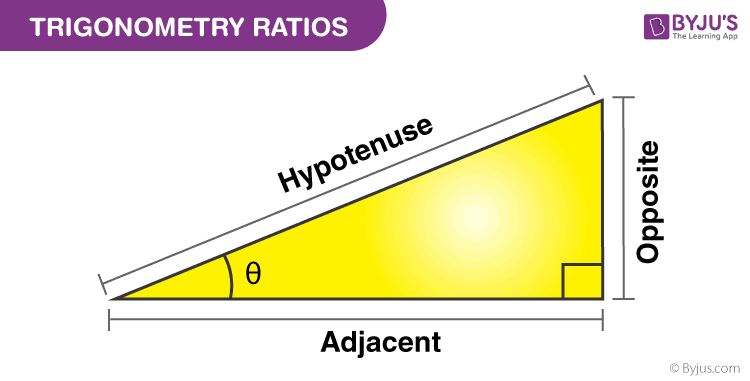## Six Important Trigonometric Functions

The six important trigonometric functions (trigonometric ratios) are calculated using the below formulas and considering the above figure. It is necessary to get knowledge about the sides of the right triangle because it defines the set of important trigonometric functions.

## Even and Odd Trigonometric Functions

The trigonometric function can be described as being even or odd.

Odd trigonometric functions: A trigonometric function is said to be an odd function if f(-x) = -f(x) and symmetric with respect to the origin.

Even trigonometric functions: A trigonometric function is said to be an even function, if f(-x) = f(x) and symmetric to the y-axis.

We know that

• Sin (-x) = – Sin x
• Cos (-x) = Cos x
• Tan (-x) = -Tan x
• Csc (-x) = – Csc x
• Sec (-x) = Sec x
• Cot (-x) = -Cot x

Therefore, cosine and secant are the even trigonometric functions, whereas sine, tangent, cosecant and cotangent are the odd trigonometric functions. If we know the even and odd trigonometric functions, it helps us to simplify the trigonometric expression when the variable inside the trigonometric function is negative.

## Trigonometry Angles

The trigonometry angles which are commonly used in trigonometry problems are  0 ° , 30 ° , 45 ° , 60 ° and 90 °. The trigonometric ratios such as sine, cosine and tangent of these angles are easy to memorize. We will also show the table where all the ratios and their respective angle’s values are mentioned. To find these angles we have to draw a right-angled triangle, in which one of the acute angles will be the corresponding trigonometry angle. These angles will be defined with respect to the ratio associated with it.

For example, in a right-angled triangle,

Sin θ = Perpendicular/Hypotenuse

or θ = sin -1  (P/H)

θ = cos -1  (Base/Hypotenuse)

θ = tan -1  (Perpendicular/Base)

## Trigonometry Table

Check the table for common angles which are used to solve many trigonometric problems involving trigonometric ratios.

In the same way, we can find the trigonometric ratio values for angles beyond 90 degrees, such as 180°, 270° and 360°.

## Unit Circle

The concept of unit circle helps us to measure the angles of cos, sin and tan directly since the centre of the circle is located at the origin and radius is 1. Consider theta be an angle then,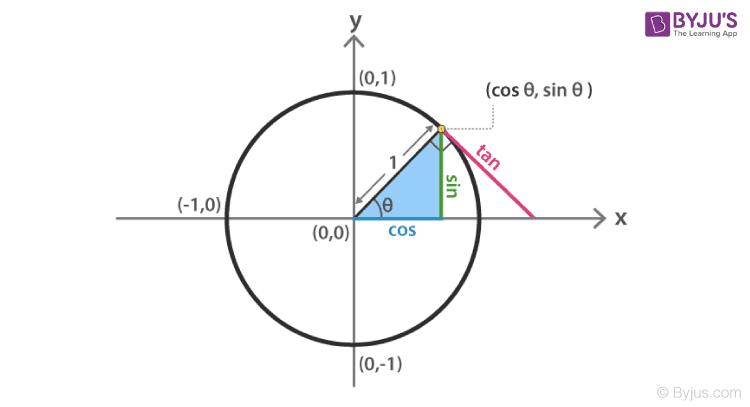Suppose the length of the perpendicular is y and of base is x. The length of the hypotenuse is equal to the radius of the unit circle, which is 1. Therefore, we can write the trigonometry ratios as;

## List of Trigonometry Formulas

The Trigonometric formulas or Identities are the equations which are true in the case of Right-Angled Triangles. Some of the special trigonometric identities are given below –

• Pythagorean Identities
• sin²θ + cos²θ = 1
• tan 2 θ + 1 = sec 2 θ
• cot 2 θ + 1 = cosec 2 θ
• sin 2θ = 2 sin θ cos θ
• cos 2θ = cos²θ – sin²θ
• tan 2θ = 2 tan θ / (1 – tan²θ)
• cot 2θ = (cot²θ – 1) / 2 cot θ
• Sum and Difference identities-

For angles u and v, we have the following relationships:

• sin(u + v) = sin(u)cos(v) + cos(u)sin(v)
• cos(u + v) = cos(u)cos(v) – sin(u)sin(v)
• $$\begin{array}{l}tan(u+v) = \frac{tan(u)\ +\ tan(v)}{1-tan(u)\ tan(v)}\end{array}$$
• sin(u – v) = sin(u)cos(v) – cos(u)sin(v)
• cos(u – v) = cos(u)cos(v) + sin(u)sin(v)
• $$\begin{array}{l}tan(u-v) = \frac{tan(u)\ -\ tan(v)}{1+tan(u)\ tan(v)}\end{array}$$
• If A, B and C are angles and a, b and c are the sides of a triangle, then,
• a/sinA = b/sinB = c/sinC

Cosine Laws

• c 2  = a 2  + b 2  – 2ab cos C
• a 2  = b 2  + c 2  – 2bc cos A
• b 2  = a 2  + c 2  – 2ac cos B

## Trigonometry Identities

The three important trigonometric identities are:

• tan² θ + 1 = sec² θ
• cot ² θ + 1 = cosec² θ

## Euler’s Formula for trigonometry

As per the euler’s formula,

e ix = cos x + i sin x

Where x is the angle and i is the imaginary number.

$$\begin{array}{l}\sin x=\frac{e^{i x}-e^{-i x}}{2 i}\\ \cos x=\frac{e^{i x}+e^{-i x}}{2}\\ \tan x=\frac{\left(e^{i x}-e^{-i x}\right)}{i\left(e^{i x}+e^{-i x}\right)}\end{array}$$

## Trigonometry Basics

The three basic functions in trigonometry are sine, cosine and tangent. Based on these three functions the other three functions that are cotangent, secant and cosecant are derived.

All the trigonometrical concepts are based on these functions. Hence, to understand trigonometry further we need to learn these functions and their respective formulas at first.

If θ is the angle in a right-angled triangle, then

Cos θ = Base/Hypotenuse

Tan θ = Perpendicular/Base

Perpendicular is the side opposite to the angle θ.

The base is the adjacent side to the angle θ.

The hypotenuse is the side opposite to the right angle

The other three functions i.e. cot, sec and cosec depend on tan, cos and sin respectively, such as:

Cot θ = 1/tan θ

Sec θ = 1/cos θ

Cosec θ = 1/sin θ

Cot θ = Base/Perpendicular

Sec θ = Hypotenuse/Base

Cosec θ = Hypotenuse/Perpendicular

## Trigonometry Examples

There are many real-life examples where trigonometry is used broadly.

If we have been given with height of the building and the angle formed when an object is seen from the top of the building, then the distance between object and bottom of the building can be determined by using the tangent function, such as tan of angle is equal to the ratio of the height of the building and the distance. Let us say the angle is ∝, then

Tan ∝ = Height/Distance between object & building

Distance = Height/Tan ∝

Let us assume that height is 20m and the angle formed is 45 degrees, then

Distance = 20/Tan 45°

Since, tan 45° = 1

So, Distance = 20 m

## Applications of Trigonometry

• Its applications are in various fields like oceanography, seismology, meteorology, physical sciences, astronomy, acoustics, navigation, electronics, etc.
• It is also helpful to measure the height of the mountain, find the distance of long rivers, etc.

## Video Lesson on Applications of Trigonometry## Trigonometry Problems and Solutions

Example 1 : Two friends, Rakesh and Vishal started climbing a pyramid-shaped hill. Rakesh climbs 315 m and finds that the angle of depression is 72.3 degrees from his starting point. How high is he from the ground?

Solution : Let m is the height above the ground.

To find: Value of m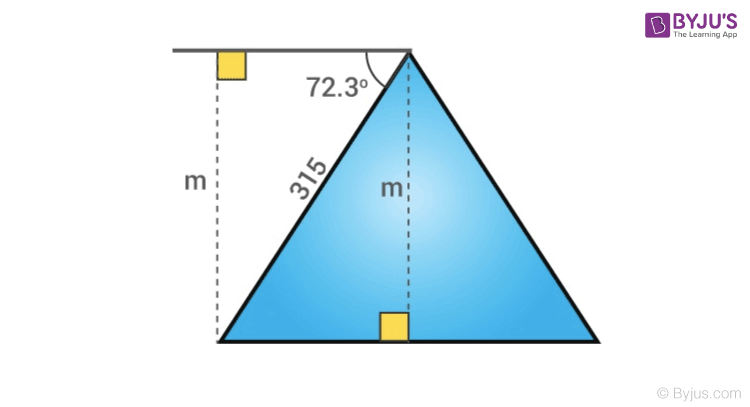To solve m, use the sine ratio.

Sin 72.3° = m/315

0.953 = m/315

m= 315 x 0.953

m=300.195 mtr

The man is 300.195 mtr above the ground.

Example 2: A man is observing a pole of height 55 foot. According to his measurement, pole cast a 23 feet long shadow. Can you help him to know the angle of elevation of the sun from the tip of shadow?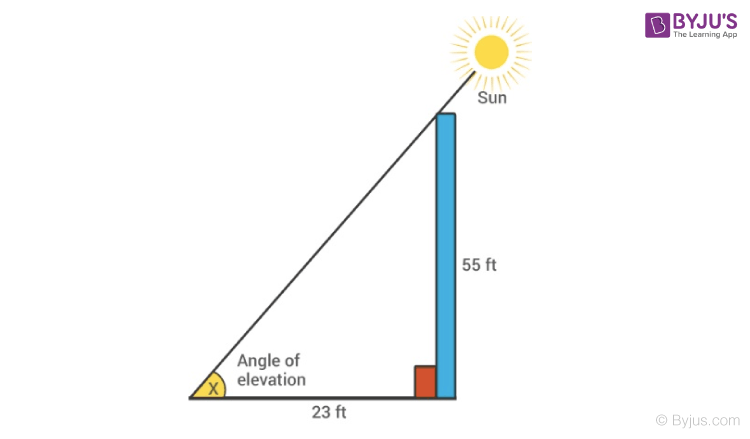Let x be the angle of elevation of the sun, then

tan x = 55/23 = 2.391

x = tan -1 (2.391)

or x = 67.30 degrees

## Trigonometry Questions

Practise these questions given here to get a deep knowledge of Trigonometry. Use the formulas and table given in this article wherever necessary.

Q.1: In △ABC, right-angled at B, AB=22 cm and BC=17 cm. Find:

(a) sin A Cos B

(b) tan A tan B

Q.2: If 12cot θ= 15, then find sec θ.

Q.3: In Δ PQR, right-angled at Q, PR + QR = 30 cm and PQ = 10 cm. Determine the values of sin  P,  cos  P  and tan  P.

## Frequently Asked Questions on Trigonometry

What do you mean by trigonometry.

Trigonometry is one of the branches of mathematics which deals with the relationship between the sides of a triangle (right triangle) with its angles. There are 6 trigonometric functions for which the relation between sides and angles are defined. Learn more about trigonometry now by visiting BYJU’S.

## What are the six basic Trigonometric Functions?

There are 6 trigonometric functions which are:

• Sine function
• Cosine function
• Tan function
• Sec function
• Cot function
• Cosec function

## What is the formula for six trigonometry functions?

What is the primary function of trigonometry, who is the founder of trigonometry, what are the applications of trigonometry in real life.

One of the most important real-life applications of trigonometry is in the calculation of height and distance. Some of the sectors where the concepts of trigonometry are extensively used are aviation department, navigation, criminology, marine biology, etc. Learn more about the applications of trigonometry here.

Learn about Trigonometry in a simple manner with detailed information, along with step by step solutions to all questions, only at BYJU’S. Download the app to get personalised videos.Put your understanding of this concept to test by answering a few MCQs. Click ‘Start Quiz’ to begin!

Select the correct answer and click on the “Finish” button Check your score and answers at the end of the quiz

Visit BYJU’S for all Maths related queries and study materials

Request OTP on Voice Call

Post My Comment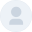It’s a detailed explanation. Helps for my kid exam preparation

Gives a very good explanation. Best for the beginner’s as well as professional

That is my very nice topic in mathematics. Am very happy to this topic tank to all mathemateciat

it’s very nice topic in mathematics.

I very happy with this topic is a very nice topic in mathematics

Very nice explain 😃

So brilliant, well explained.

Best way of teaching Keep it up• Share Share

Register with byju's & watch live videos.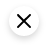## Trig Calculator

The sine and cosine trigonometric functions, tan, cot, sec, and csc, calculated from trig identities., trigonometry in a right triangle, other trigonometric calculators.

Welcome to this trigonometric calculator, a trig tool created to:

• Calculate any trigonometric function by inputting the angle at which you want to evaluate it; and
• Solve for the sides or angles of right triangles by using trigonometry.

Trig functions are functions that take an angle as the argument. We define these functions by using the angle of a right triangle that is inserted in a unitary circle . Then, we relate that angle to the sides of such a triangle.

As the right triangle is circumscribed in a unit circle, the length of its hypotenuse equals the circle's radius (which equals one unit).

Sine and cosine are the fundamental trigonometric functions arising from the previous diagram:

• The sine of theta ( sin θ ) is the hypotenuse's vertical projection (green line); and
• The cosine of theta ( cos θ ) is the hypotenuse's horizontal projection (blue line).

We can rotate the radial line through the four quadrants and obtain the values of the trig functions from 0 to 360 degrees , as in the diagram below:

• For example, for an angle that leads to the second quadrant (90-180°), the cosine will be negative as the horizontal projection of the hypotenuse will point to the left. That's the case of 135°, whose cosine (horizontal projection, or x coordinate) equals -√2/2.
• For the third quadrant (180-270°), the cosine and the sine of the angles lying on it will be negative.
• For the fourth quadrant angles (270-360)°, the cosines will be positive and the sines negative.## Beyond 360 degrees

The previous behavior repeats cyclically, so trigonometric functions are not limited to 360°. We can keep rotating counterclockwise, and once we reach 360 degrees, the sine and cosine functions start to repeat the same behavior. As a consequence, we can relate the functions at different angles with the following trig identities for any n integer:

• sin(θ + 2πn) = sin(θ) ;
• cos(θ + 2πn) = cos(θ); and

For example a trig function at 90° (π/2) will be mathematically the same as at 450° (5π/2), as 5π/2 = π/2 + 2π.

## Negative angles

Negative angles imply the same way to calculate sine and cosine (vertical and horizontal projections, respectively), with the difference that angular rotation occurs in the clockwise direction. For example, a trigonometric function at 270° is the same as at -90°, as their radial lines are the same (you can check it with this calculator)

Once you know the value of sine and cosine, you can use the following trigonometric identities to obtain the values of the other four functions:

Tangent is the sine-to-cosine ratio

tan(α) = sin(α)/cos(α)

Cosecant is the reciprocal of the sine

csc(α) = 1/sin(α)

Secant is the reciprocal of the cosine

sec(α) = 1/cos(α)

Cotangent is the reciprocal of the tangent

cot(α) = 1/tan(α)

From the previous analysis, we can obtain some valuable formulas that relate the angle of a right triangle to its sides. Of course, this doesn't limit to unit circles, so we can use it for hypotenuses of any length.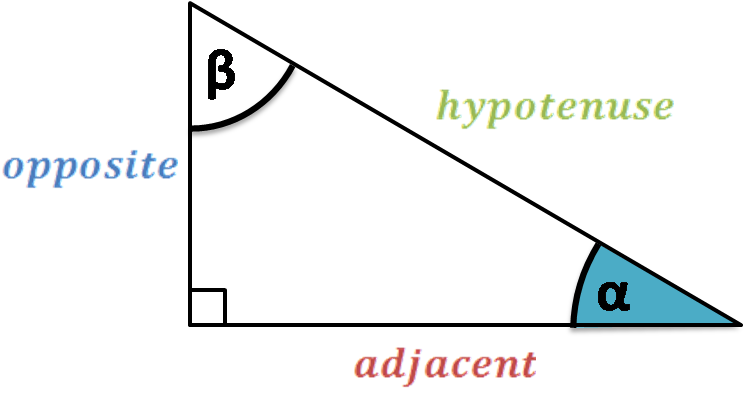We relate the angle of the right triangle to its sides in the following way:

sin(α) = opposite/hypotenuse

Remember that cotangent, secant, and cosecant are the inverse of the previous functions:

csc(α) = 1/sin(α) = hypotenuse/opposite

You also can apply the previous formulas for the other acute angle ( β ), but consider that the legs of the triangle will switch: the adjacent will now be the hypotenuse and vice versa.

If you like this calculator, you may find these other tools interesting:

• Trigonometry calculator ;
• Cosine triangle calculator ;
• Sine triangle calculator ;
• Trig triangle calculator ;
• Right triangle trigonometry calculator ;
• Sine cosine tangent calculator ;
• Tangent ratio calculator ; and
• Tangent angle calculator .

## How do I solve a 45-90-45 degree triangle by trig formulas?

If one leg of a 45 45 90 triangle is equal to a , then:

• The second leg also equals a ;
• The hypotenuse equals a √2 (from the hypotenuse formula c = √( a ² + a ²) = a √2 );
• The area is A = a ²/2 ; and
• The perimeter equals a (2 + √2) (the sum of the two sides plus the hypotenuse).

## What are the values of the 6 trig functions at 90 degrees?

The values of the 6 trig functions for 90 degrees (π/2) are the following ones:

• sin(90°) = 1;
• cos(90°) = 0;
• tan(90°)= undefined;
• cot(90°) = 0;
• sec(90°) = undefined; and
• csc(90°) = 1.

## Circle skirt

Humans vs vampires, relative change.

• Biology ( 97 )
• Chemistry ( 96 )
• Construction ( 139 )
• Conversion ( 257 )
• Ecology ( 27 )
• Everyday life ( 251 )
• Finance ( 551 )
• Food ( 65 )
• Health ( 436 )
• Math ( 656 )
• Physics ( 497 )
• Sports ( 101 )
• Statistics ( 182 )
• Other ( 173 )
• Discover Omni ( 40 )
• Pop culture
• Writing tips
• Daily Crossword
• Word Puzzle
• Word Finder
• Word of the Day
• Synonym of the Day
• Word of the Year
• Language stories
• All featured
• Gender and sexuality
• All pop culture
• Grammar Coach ™
• Writing hub
• Grammar essentials
• Commonly confused
• All writing tips

trigonometry .

## Origin of trig

Words nearby trig.

• trifunctional
• trigeminal nerve

## Other definitions for trig (2 of 4)

neat, trim, smart, or spruce.

in good physical condition; sound; well.

to make trim, smart, etc. (often followed by up or out ).

## Other words for trig

Other words from trig.

• trigness, noun

## Other definitions for trig (3 of 4)

to support or prop, as with a wedge.

to act as a check on (the moving of wheels, vehicles, etc.).

a wedge or block used to prevent a wheel, cask, or the like, from rolling.

## Other definitions for trig. (4 of 4)

trigonometric.

trigonometrical.

trigonometry.

Dictionary.com Unabridged Based on the Random House Unabridged Dictionary, © Random House, Inc. 2023

## How to use trig in a sentence

“I think in regard to trig , anything is possible,” McGinniss writes.

Palin dedicates the book to her 2-year old son, trig , who has Down syndrome.

The media frenzy around them was astonishing—they were rock stars, from Bristol and Levi down to little trig .

How could I ever forget the sight of a banner held up high to say “We Are Here For trig ”?

Has Senator McCain simply forgotten that Calculus Algebra trig has Down Syndrome?

Jane was a trig , neat body; small, as the coast girls often are—I wonder why?

Harry and Seth made everything trig and came in last, leaving the cat-boat at anchor far out.

The trig little sail-boat turned out to be a respectable yacht, steam, at that.

trig ′amist, one who marries three wives; trig ′amy, the state of having three husbands or wives at the same time.

Trigla, trig ′la, n. the typical genus of Triglid, the gurnards.

## British Dictionary definitions for trig (1 of 3)

/ ( trɪɡ ) archaic , or dialect /

neat or spruce

to make or become trim or spruce

## Derived forms of trig

• trigness , noun

## British Dictionary definitions for trig (2 of 3)

/ ( trɪɡ ) mainly dialect /

a wedge or prop

to block or stop

to prop or support

## British Dictionary definitions for trig. (3 of 3)

trigonometrical

trigonometry

Collins English Dictionary - Complete & Unabridged 2012 Digital Edition © William Collins Sons & Co. Ltd. 1979, 1986 © HarperCollins Publishers 1998, 2000, 2003, 2005, 2006, 2007, 2009, 2012

## This Educator Uses Coding and SEL to Make Math More Engaging• Share article

Math skills, such as data analysis and statistics , are one of the most sought-after skills for new employees, even in fields outside of STEM. But many students think that they’re not a “math person” and disengage from math topics.

In addition, students’ math scores hit historically low levels on national assessments during the pandemic, and educators are looking for ways to engage their students in the subject.

Lindsay Gold, an associate professor in the school of education at the University of Dayton in Ohio, and one of her undergraduate students wanted to find out if incorporating coding and social-emotional learning into math lessons would increase elementary students’ interest in math.

“There are other ways to teach math that are not stress-induced and pressure-forming, because we want the students to see that math is fun,” Gold said. “We don’t want it to be the subject that everybody hates and dreads.”

In an interview with Education Week, Gold explained how she integrated coding and SEL principles into 3rd, 4th, and 5th grade math lessons and how it affected student engagement.

This conversation has been edited for brevity and clarity.

## How did you incorporate coding into elementary math lessons?To introduce coding principles, we talked about the engineering design process. It was a basic version of the “ask, imagine, plan, create, and improve” process. Then students had to write what their idea of coding was. Some drew pictures, some wrote up sentences. A lot of the things they could think of had to do with video games and that kind of stuff. We also read technical writing to be able to think about different coding languages.

Then using Texas Instruments’ TI-Nspire [a graphing calculator] and TI Innovator Rover [a robotic vehicle], we taught students to code for sound and color. First, we had students get used to what happens when you input a code [in the graphing calculator]. For instance, they would enter a value for red, green, or blue, and see what color it makes together. Then they can go back and manipulate [the values] to change the color. We did the same thing for sound. We talked about frequency and pitch. And then we got into the geometry aspect of it, coding for lines, segments, arrays. We asked them to code a line segment that was X centimeters long. And then we did the same thing with angles, rectangles, triangles, pentagons, etcetera.

Once we got through that portion, we had a track challenge for those who were comfortable. We taped off a track on the floor and they had to see if they could completely write their own program to get the rover to go through the track. We purposely put in angles and things that we just talked about into the track to see if they could do that. Everybody was successful, and they all got their driver’s license to drive the TI Rover.## How was social-emotional learning added to the mix?

SEL was a big part of it. We wanted to get students to work together, to be self-aware, to be aware of others, to get confidence in providing their own opinion, and to work on the ability to listen to others. We wanted the students to feel like everybody had a voice, and that doesn’t always happen in the mathematics classroom. There tends to be this hierarchy where students either feel really confident and comfortable in their ability, or they feel very much like they’re not a math person. We have to talk a lot about there’s no such thing as a math person. It’s not the secret club that you weren’t invited to. [SEL] showed the students that everybody had something to offer.

## What did teachers think of this activity?

In the beginning, they were really hesitant. They were very nervous about the calculator and extremely nervous about coding because they didn’t have experience with that. But they saw that their elementary students could do it, and that they could use this technology to enhance instruction. I wanted to show them that there are ways to teach math and engage in math that students will remember and internalize and want to do more. When we do this again, we’re going to do even more teacher professional development to make sure that they’re comfortable.

## How did this activity affect student engagement and performance?

They very much enjoyed the coding, and they very much enjoyed having the ability to make something work. They felt more comfortable with the concepts, in their abilities to do the math, to engage in STEAM [science, technology, engineering, art, and math] learning, they better understood the engineering design process. And while we couldn’t track actual student achievement to see if there was a correlation there, we were able to see that in the spring, when the teachers talked about angles again, the students remembered what we had done in the fall. Projects like this are teaching students how to really problem-solve and think versus just memorize.

Edweek top school jobs.Supported by

## Gen Alpha Is Here. Can You Understand Their Slang?

Are you a “sigma”? Do you have “rizz”? The youngest generation is bamboozling its elders with terms all their own.Do you know what a gyat is? What about a rizzler? And how, precisely, does one pay a Fanum tax?

Welcome to the language of Gen Alpha, the cohort coming up right behind Gen Z. These children of millennials have begun a generational rite of passage — employing their own slang terms and memes, and befuddling their elders in the process.

Which brings us back to gyat (rhymes with “yacht,” with a hard “g” and a firm emphasis on “yat”).

“There’s no cute way to say it — it’s just a word for a big butt,” said Alta, a 13-year-old eighth grader in Pennsylvania. “If someone has a big butt, someone will say ‘gyat’ to it.”

Alta and her brother Kai, an 11-year-old sixth grader, said they had learned the word on TikTok and that it had suddenly become popular among their classmates. The internet encyclopedia Know Your Meme credits the sudden popularity of “gyat” to the Twitch livestreamer Kai Cenat. (In August, Mr. Cenat made headlines when his fans swarmed Union Square Park in Manhattan after he promised to give away gaming consoles at no cost.)

“I don’t say ‘gyat’ to people, though, unless they’re my friend,” Alta said. “And we say it to our mom.”

Several other new words have become part of this generation’s vernacular, and six members of Gen Alpha offered their decoding services for this article. (Their parents gave permission for them to be interviewed, with the agreement that their last names would not be used.) Many of the children cited a catchy parody song making the rounds on TikTok as a key to the slang’s rising popularity. The lyrics go like this:

Sticking out your gyat for the rizzler

You’re so skibidi

You’re so Fanum tax

I just wanna be your sigma

A rizzler is a “good person,” according to Malcolm, a 10-year-old in Washington state.

“Having rizz is when you have good game,” Alta said. “Being a rizzler is like when you’re a pro at flirting with people.” (Rizz is short for charisma.)

The word can be used as a compliment or a joke, according to Jaedyn, 12. She said that the boys at her school in New Jersey had been singing the song lately, adding that it gave her a headache.

Jaedyn added that “nobody really knows” the meaning of “skibidi.” It has entered the lexicon by way of the animated series “ Skibidi Toilet ,” which has racked up more than 700 million views on YouTube. A typical episode is about 15 seconds long and features a man who pops his head out of a toilet bowl and launches into a song heavy on the use of the word that gives the show its name. (It’s easier if you just watch it . Boomers might think of “Skibidi Toilet” as a 2020s answer to the animations of “Monty Python’s Flying Circus.”)

“I don’t like,” Tariq, 8, said of the series. “It creeps me out. Every time I go to the toilet, I just want to get it quick done.” Tariq, who lives in New York State and is known online as Corn Kid , said he was not familiar with the other terms.

Fanum tax refers to Fanum, a popular streamer on Twitch who regularly appears online with Mr. Cenat. When friends are eating in Fanum’s presence, he insists that they share some of their food with him. That’s the Fanum tax.

And sigma has something to do with wolves.

“Everyone in my grade, at least, says it in a way where they’re like the alpha of the pack,” Alta said. “If you’re trying to say you’re dominant and you’re the leader, you’ll call yourself ‘sigma.’”

In a TikTok video posted in October, Philip Lindsay, a special-education math teacher in Payson, Ariz., listed a few terms he had been hearing in the classroom, including Fanum tax and gyat. “Which does not mean ‘get your act together,’” Mr. Lindsay, 29, said in the video , which has since been viewed over four million times.

His students tried at first to make him believe that gyat was an acronym that stood for “go you athletic team,” he said in an interview. He recently had to explain gyat’s real meaning to a colleague whose students had convinced the teacher to display the word in the classroom.

Mr. Lindsay said the new words struck him as more “meme-like” than earlier slang terms. He added that he believed they were “driven mainly by social media, TikTok specifically.”

Gen Alpha is still being born, according to demographers. Its birth years span from 2010 to 2025, said Mark McCrindle, a generational researcher in Australia who coined the name Gen Alpha several years ago.

Online, members of Gen Z have begun to realize they are no longer the new kids on the digital block — and that Gen Alpha might be coming for them, in the same way that they had once gone after millennials.

Anthony Mai, a TikTok creator with a large following, recently posted a video of himself wearing a comically deadpan expression as the Gen Alpha-slang song played. “Gen Alpha is making their own memes now,” he wrote in a caption. “It has begun. We are the next cringe gen on the chopping block.”

Intergenerational comedy has become a staple on social media platforms, where creators dramatize the differences between age groups. Skibidi and gyat fit snugly into the memes and video shorts belonging to this subgenre.

“Whenever I think about the linguistic differences between generations, I just think, Are we really going to do this again?” said Jessica Maddox, an assistant professor of digital media at the University of Alabama. “Generational differences and divides have always been played up to some extent, even before the heyday of the internet, but social media really exacerbates them.” She cited “ OK, boomer ,” a retort popularized online by Gen Z in 2019, as an example.

As Gen Alpha’s slang terms make their way into the wider (read: older) world, the young people responsible for their popularity are ready to move onto what’s next.

“If millennials start saying them, we’ll be like, ‘We’re done with these now,’” Jaedyn said.

Madison Malone Kircher is a reporter for The Times. She writes about the internet for the Styles desk. More about Madison Malone Kircher

## A Guide to Aging Well

Looking to grow old gracefully we can help..

Your body’s need for fuel shifts as you get older. Your eating habits should shift , too.

Older people are using cannabis more than ever. Here’s what to know about the potential medicinal benefits and the side effects .

The sun’s rays cause the majority of skin changes as you grow older. Here’s how sunscreen helps prevent the damage .

Joint pain, stiffness and swelling aren’t always inevitable results of aging, experts say. Here’s what you can do to reduce your risk for arthritis .

Getting older is inevitable (and certainly better than the alternative). Here's how to keep your body tuned up and your mind tuned in .

Memory decline is not inevitably tied with aging. Here are a few simple things you can do to keep you sharp .

BREAKING: Former first lady Rosalynn Carter dies at 96

## Snoop Dogg says he’s giving up smoking after years of marijuana useKnown weed enthusiast and rapper Snoop Dogg announced the unthinkable on Thursday — he’s giving up smoking.

The hip-hop star, whose real name is Calvin Broadus, did not specify what kind of smoke he’s quitting — or even whether he’s serious.

“After much consideration & conversation with my family, I’ve decided to give up smoke. Please respect my privacy at this time,” he posted on Instagram and X .

In replies to Snoop's post on X, some fans met the news with a healthy dose of skepticism.

"Today isn’t April Fools day Snoop," one replier commented.

Another person on X responded that he thought Snoop's announcement was a smoke screen and that the rapper was just transitioning to edibles to give his lungs a break.

"He’s moving to edible’s letting his lungs rest a little eat up champ."

On Instagram, one Snoop follower appeared to take his message seriously: "I had a pulmonary embolism last year and had to give up smoking as well; so more edibles for Edible Dee it is; but i know how hard this transition is especially for someone like you. Take all the time you need."

Another poster on Instagram praised Snoop for announcing publicly that he had quit, considering all the money he's made while openly promoting marijuana. Images of Snoop with a blunt in his hand are ubiquitous on the internet.

"The courage, the boldness, the ability to announce that you’re stopping something that made you SO SO SO much money which created a character that you and all of us are used too is truly remarkable," the poster wrote. "As a sober man I speak for me and all of us sober people around the world, welcome to sobriety!!!"

Representatives for Snoop Dogg were not immediately reached for comment Thursday.

Snoop and marijuana have been inextricably linked during a career spanning decades.

He rose in popularity after he made several guest appearances on Dr. Dre's 1992 album, "The Chronic." Art on the CD was emblazoned with a marijuana leaf.

Snoop released his debut album, "Doggystyle," in 1993, which quickly made him a household name and cemented him as a top-tier rapper.

Snoop also made a cameo on the 1998 ﻿cult hit "Half Baked," about a group of friends who are potheads, which starred Dave Chappelle.

Antonio Planas is a breaking news reporter for NBC News Digital.#### IMAGES

1. Unit Circle Trig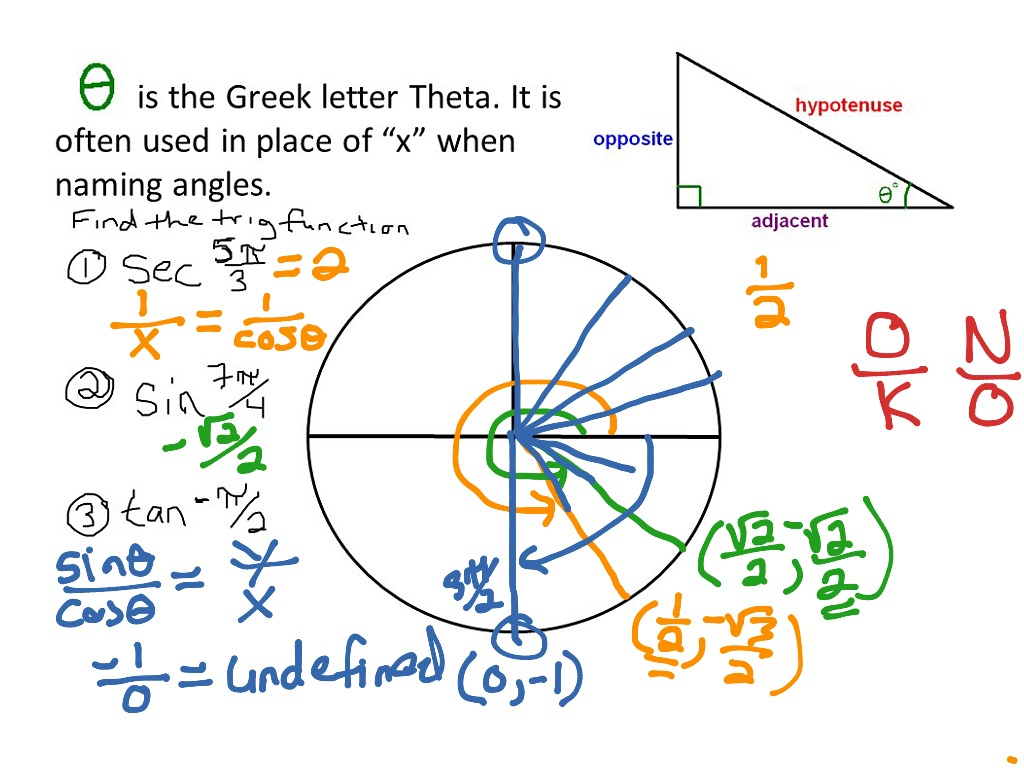2. Trigonometry Formulas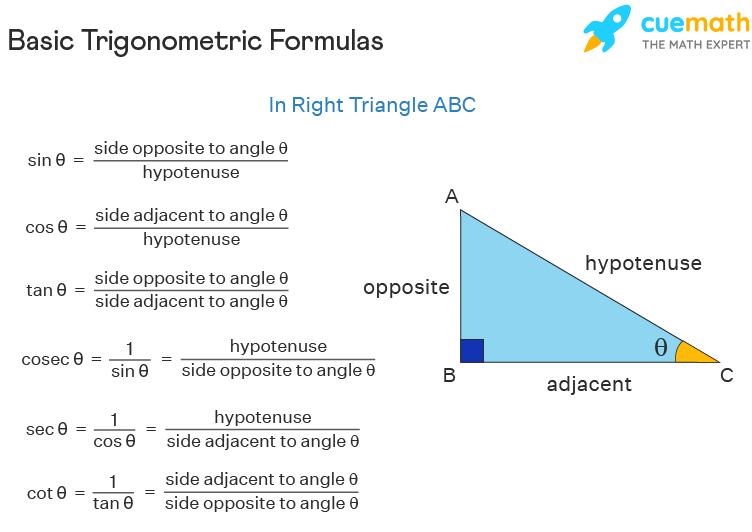3. Introduction to Trigonometric Functions Using Triangles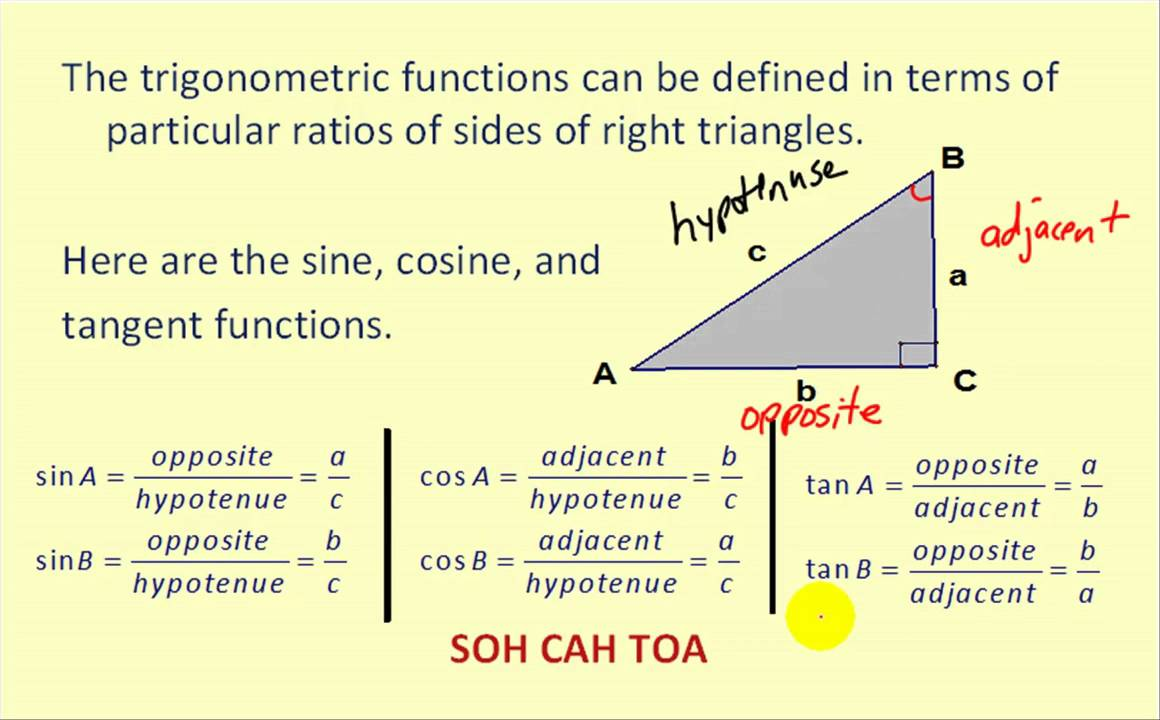4. Lesson 5: Right Triangle Trigonometry. Trig ratios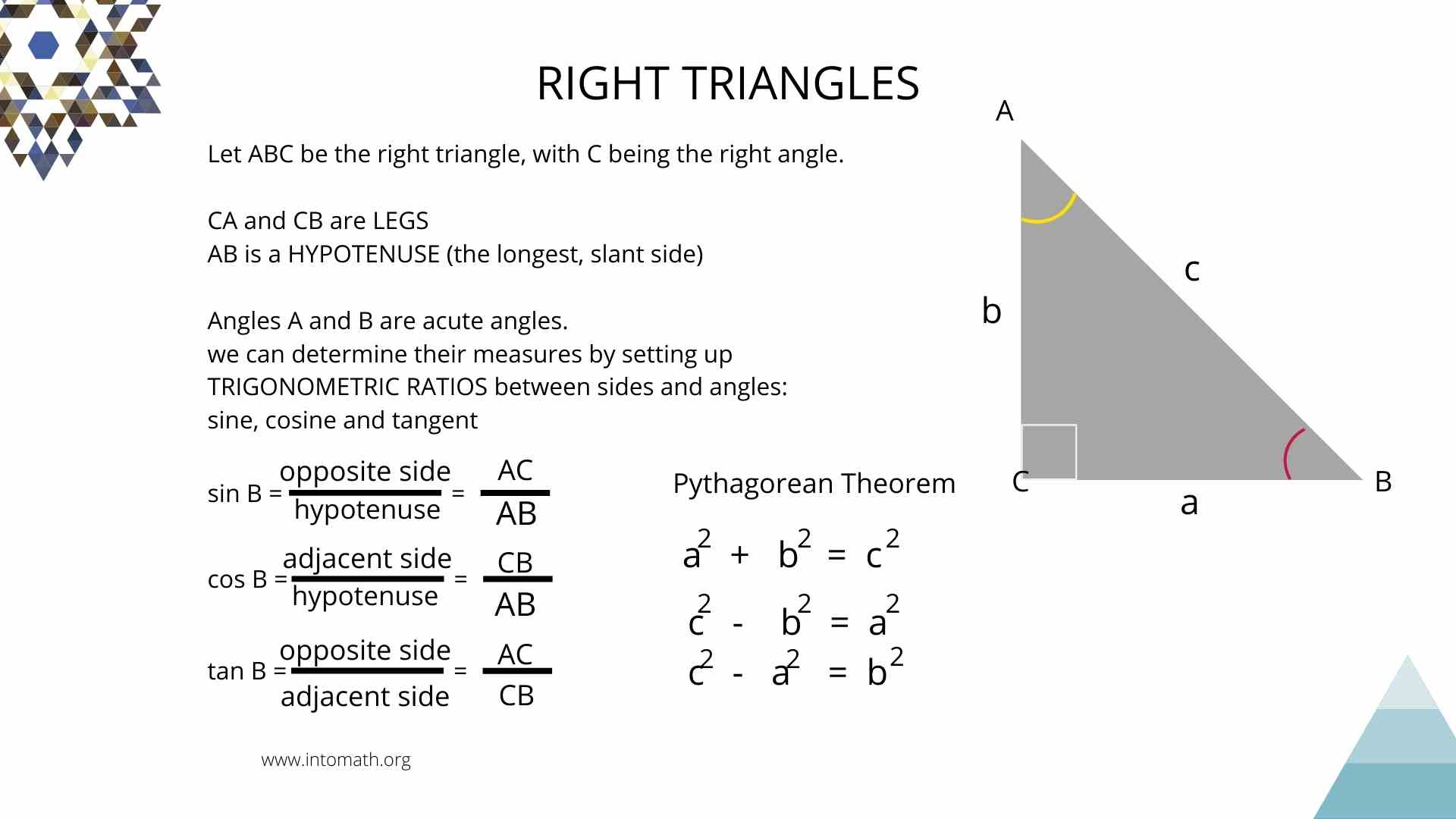5. Trigonometry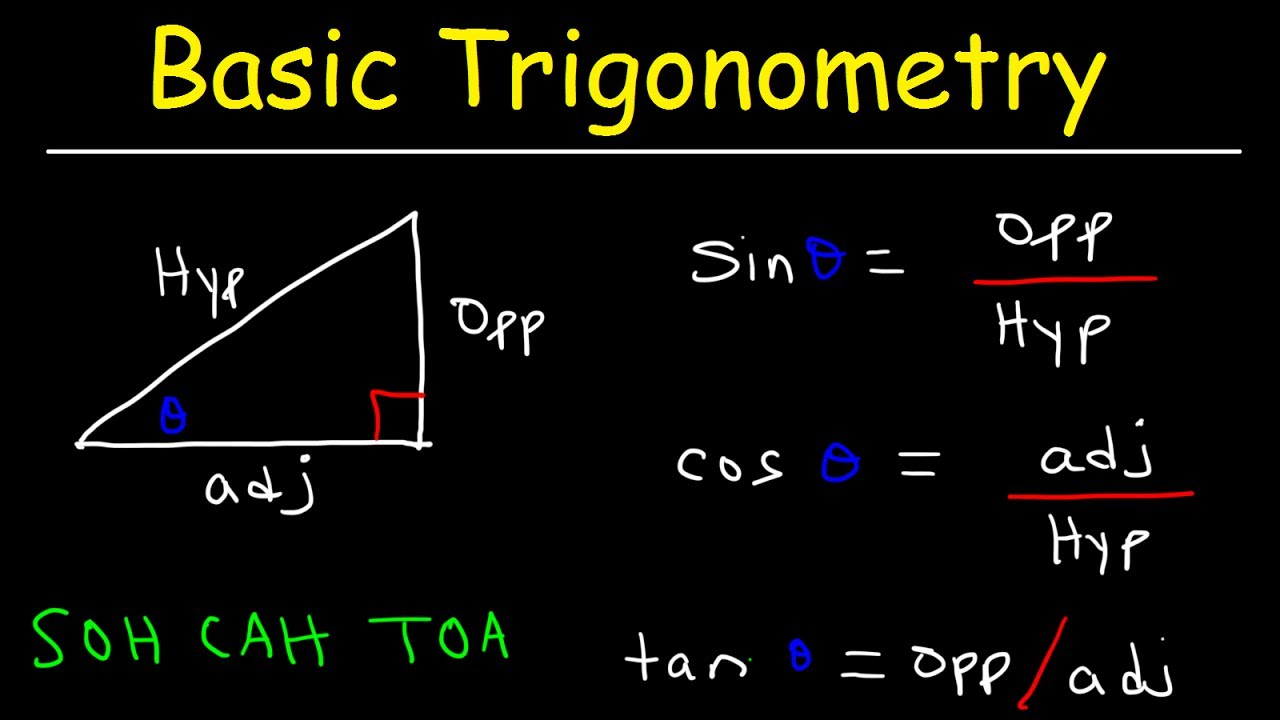6. Trig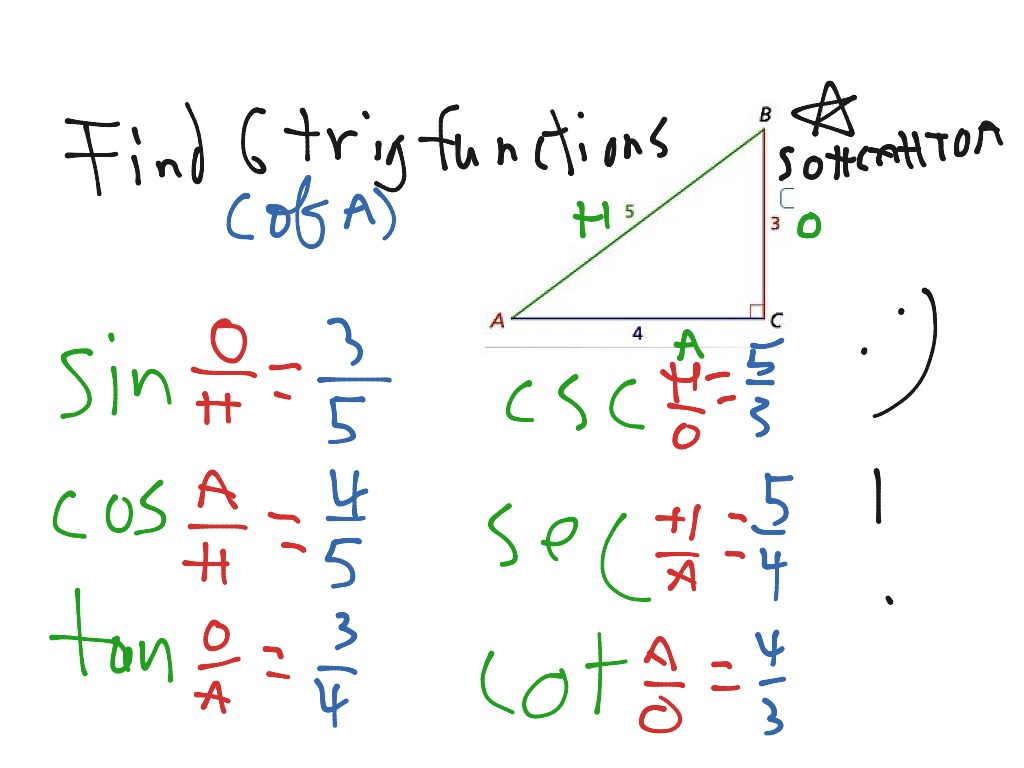#### VIDEO

1. MATH 220 Week 9 Trig Subs Example 3

2. Trigonometric function |#mathtrick |#shorts |#mathformulas |#trigonometry

3. Kindergarten Math With a Teacher- Math Daily 3

4. Trig Ratios Review in Under 60 Seconds! #Shorts #trig #trigonometry #math #learn

5. MATH Session 3 TRIG

6. 45 45 90 TRIANGLE-( Find Side Length )

1. Sine, Cosine, Tangent

Exercise. Try this paper-based exercise where you can calculate the sine function for all angles from 0° to 360°, and then graph the result. It will help you to understand these relatively simple functions. You can also see Graphs of Sine, Cosine and Tangent.. And play with a spring that makes a sine wave.. Less Common Functions. To complete the picture, there are 3 other functions where we ...

2. Introduction to Trigonometry

Trigonometry helps us find angles and distances, and is used a lot in science, engineering, video games, and more! Right-Angled Triangle. The triangle of most interest is the right-angled triangle. The right angle is shown by the little box in the corner: Another angle is often labeled θ, and the three sides are then called:

3. Trigonometry

Trigonometry (from Ancient Greek τρίγωνον (trígōnon) 'triangle', and μέτρον (métron) 'measure') is a branch of mathematics concerned with relationships between angles and ratios of lengths. The field emerged in the Hellenistic world during the 3rd century BC from applications of geometry to astronomical studies. The Greeks focused on the calculation of chords, while ...

4. Trigonometric functions

Basis of trigonometry: if two right triangles have equal acute angles, they are similar, so their side lengths are proportional.. In mathematics, the trigonometric functions (also called circular functions, angle functions or goniometric functions) are real functions which relate an angle of a right-angled triangle to ratios of two side lengths. They are widely used in all sciences that are ...

5. Trigonometric functions

Algebra (all content) 20 units · 412 skills. Unit 1 Introduction to algebra. Unit 2 Solving basic equations & inequalities (one variable, linear) Unit 3 Linear equations, functions, & graphs. Unit 4 Sequences. Unit 5 System of equations. Unit 6 Two-variable inequalities. Unit 7 Functions. Unit 8 Absolute value equations, functions, & inequalities.

6. Trigonometry

Trigonometry, the branch of mathematics concerned with specific functions of angles. There are six functions commonly used in trigonometry: sine (sin), cosine (cos), tangent (tan), cotangent (cot), secant (sec), and cosecant (csc). Learn more about trigonometry in this article.

7. Trigonometry

Trigonometry 4 units · 36 skills. Unit 1 Right triangles & trigonometry. Unit 2 Trigonometric functions. Unit 3 Non-right triangles & trigonometry. Unit 4 Trigonometric equations and identities.

8. Trigonometry

Level up on all the skills in this unit and collect up to 1700 Mastery points! Let's extend trigonometric ratios sine, cosine, and tangent into functions that are defined for all real numbers. You might be surprised at how we can use the behavior of those functions to model real-world situations involving carnival rides and planetary distances.

9. Trigonometry: FAQ (article)

Learn for free about math, art, computer programming, economics, physics, chemistry, biology, medicine, finance, history, and more. Khan Academy is a nonprofit with the mission of providing a free, world-class education for anyone, anywhere. ... Trigonometric functions are used in many real-world applications. For example, engineers use them to ...

10. Trigonometric functions

Level up on all the skills in this unit and collect up to 1900 Mastery points! Start Unit test. Discover how to measure angles, distances, and heights using trigonometric ratios and the unit circle. Learn how to use sine, cosine, and tangent to solve real-world problems involving triangles and circular motion.

11. Calculus I

In this section we will give a quick review of trig functions. We will cover the basic notation, relationship between the trig functions, the right triangle definition of the trig functions. We will also cover evaluation of trig functions as well as the unit circle (one of the most important ideas from a trig class!) and how it can be used to evaluate trig functions.

12. Trigonometric Functions

6. Trigonometric Functions of Any Angle - don't give up in this section! 7. Radians - the alternative to degrees - and much more useful. 8. Applications of Radian Measure - includes arc length, area of a sector, angular velocity, a game and pulleys. 9. Radians and the Trigonometric Ratios - how trigonometry works when the angle measure is radians.

13. Finding a Rare Use for Trigonometry After High School

Trig persists as an important area of study in math class, though the occasions for it—like when you need to find the angles in a triangle based on its sides—are rather limited in daily life.

14. Right triangles & trigonometry

Learn. Getting ready for right triangles and trigonometry. Hypotenuse, opposite, and adjacent. Side ratios in right triangles as a function of the angles. Using similarity to estimate ratio between side lengths. Using right triangle ratios to approximate angle measure. Right triangles & trigonometry: FAQ.

15. Trigonometric Functions (Definition, Graphs, Solution, and Examples)

Trigonometric Functions. Trigonometric functions are also known as Circular Functions can be simply defined as the functions of an angle of a triangle. It means that the relationship between the angles and sides of a triangle are given by these trig functions. The basic trigonometric functions are sine, cosine, tangent, cotangent, secant and ...

16. Trigonometry

Trigonometry is the branch of mathematics that deals with the study of the relationship between the sides of a triangle (right-angled triangle) and its angles. The relationship is presented as the ratio of the sides, which are trigonometric ratios. The six trigonometric ratios are sine, cosine, tangent, cotangent, secant, and cosecant.

17. Trigonometric functions

Trigonometric functions are functions related to an angle. There are six trigonometric functions: sine, cosine, tangent and their reciprocals cosecant, secant, and cotangent, respectively. Sine, cosine, and tangent are the most widely used trigonometric functions. Their reciprocals, though used, are less common in modern mathematics.

18. Trigonometric Functions

Trigonometric Functions. Trigonometric functions are the basic six functions that have a domain input value as an angle of a right triangle, and a numeric answer as the range.The trigonometric function (also called the 'trig function') of f(x) = sinθ has a domain, which is the angle θ given in degrees or radians, and a range of [-1, 1].

19. Trig Definition & Meaning

The meaning of TRIG is trigonometry. How to use trig in a sentence. trigonometry… See the full definition. Games & Quizzes; Games & Quizzes; Word of the Day; Grammar; Wordplay; Word Finder ... 25 Aug. 2017 Spanish II honors will be offered at HMS, and algebra II/trig honors be offered at CHMS, ...

20. Trigonometry (Functions, Table, Formulas & Examples)

Trigonometry is one of the most important branches in mathematics that finds huge application in diverse fields. The branch called "Trigonometry" basically deals with the study of the relationship between the sides and angles of the right-angle triangle. ... Even trigonometric functions: A trigonometric function is said to be an even ...

Trigonometry is a branch of mathematics that studies relationships between side lengths and angles of triangles. In this video we look at the use of trigono...

22. Trig Calculator

Tan, cot, sec, and csc, calculated from trig identities. Once you know the value of sine and cosine, you can use the following trigonometric identities to obtain the values of the other four functions: Tangent is the sine-to-cosine ratio. tan (α) = sin (α)/cos (α) Cosecant is the reciprocal of the sine. csc (α) = 1/sin (α)

23. Trigonometry For Beginners!

This math video tutorial provides a basic introduction into trigonometry. It covers trigonometric ratios such as sine, cosine, and tangent. It explains how...

24. TRIG Definition & Usage Examples

Trig definition: . See examples of TRIG used in a sentence.

25. This Educator Uses Coding and SEL to Make Math More Engaging

This Educator Uses Coding and SEL to Make Math More Engaging. By Lauraine Langreo — November 14, 2023 4 min read. E+/Getty. Math skills, such as data analysis and statistics , are one of the ...

26. Can You Understand Gen Alpha's Slang?

As Gen Alpha's slang terms make their way into the wider (read: older) world, the young people responsible for their popularity are ready to move onto what's next. "If millennials start ...

27. Snoop Dogg says he's giving up smoking after years of marijuana use

By Antonio Planas. Known weed enthusiast and rapper Snoop Dogg announced the unthinkable on Thursday — he's giving up smoking. The hip-hop star, whose real name is Calvin Broadus, did not ...

28. To mark your child's progress, look beyond their grades, report urges

In math, just 36% of fourth-graders and 26% of eighth-graders were "proficient" in 2022. ... Teachers use a student's performance on in-class assignments and tests, their own observations ...